• 关于匹配滤波器原理以及其matlab实现方式的详细过程 关于匹配滤波器原理以及其matlab实现方式的详细过程
• 背景   雷达接收机在接收回波信号的同时，不可避免地会遇到噪声，同时还会受到各种干扰，如各种分布物体产生的杂波干扰、敌方施放的噪声调制干扰等。为了选出有用目标，同时抑制各种噪声和干扰，...匹配滤波器是在
文章目录1.背景2.数字信号接收等效原理图3.匹配滤波器的基本概念4.匹配滤波器的频率响应函数5.匹配滤波器的脉冲响应函数6.匹配滤波器的输出信号7.匹配滤波器的输出噪声8.线性调频信号的匹配滤波输出推导8.1 时域分析8.2 频域分析8.3 再变换回时域8.4 匹配滤波脉压输出性能分析9.线性调频信号脉冲压缩的匹配滤波实现9.1 时域匹配滤波9.2 频域匹配滤波9.2.1 方法一9.2.2 方法二9.2.3 方法三10.MATLAB仿真10.1 线性调频信号的匹配滤波器输出10.2 利用匹配滤波器对回波信号进行脉冲压缩来测距
1.背景
雷达接收机在接收回波信号的同时，不可避免地会遇到噪声，同时还会受到各种干扰，如各种分布物体产生的杂波干扰、敌方施放的噪声调制干扰等。为了选出有用目标，同时抑制各种噪声和干扰，需要滤波器做出频率选择，滤波器是完成这一任务的重要器件。滤波器的频带宽度和频率特性影响滤波效果，直接关系到雷达接收机的灵敏度、波形失真等重要指标。对应于不同的输入信号和噪声干扰，为了使接收机输出端的信号噪声比最大，波形失真最小，要求滤波器有一个最佳的频带宽度和频率特性形状，以实现最佳滤波。
所谓最佳是在某种准则下系统性能达到最佳。在数字通信中，最常采用的最佳准则是输出信噪比最大准则和差错概率最小准则。
最佳线性滤波器的设计通常有两种准则：

使滤波器输出的信号波形与发送信号波形之间的均方误差最小——维纳滤波器；
使滤波器输出信噪比在某一特定时刻达到最大——匹配滤波器。

2.数字信号接收等效原理图
解调器中抽样判决以前各部分电路可以用一个线性滤波器来等效，如图所示：这里，$n(t)$为高斯白噪声，均值为 0，方差为 $\sigma^2$，双边功率谱密度为 $\frac{n_0}{2}$
抽样判决器输出数据正确与否，与滤波器输出信号波形和发送信号波形之间的相似程度无关，也即与滤波器输出信号波形的失真程度无关；只取决于抽样时刻信号的瞬时功率与噪声平均功率之比，即信噪比
3.匹配滤波器的基本概念
匹配滤波器是在白噪声背景中检测信号的最佳线性滤波器，其输出信噪比在抽样判决时刻$t=t_0$可以达到最大。

4.匹配滤波器的频率响应函数
匹配滤波器的输入信号 $r(t)=s(t)+n(t)$
匹配滤波器的输出信号 $y(t)=s_o(t)+n_o(t)$
其中，根据傅里叶反变换，$s_o(t)=\frac{1}{2\pi}\int_{-∞}^{∞} {\color{Maroon}S_o(\omega)}e^{j\omega t}\,d\omega=\frac{1}{2\pi}\int_{-∞}^{∞} {\color{Maroon}S(\omega)H(\omega)}e^{j\omega t}\,d\omega$
在$t=t_0$时刻信号的瞬时功率：
$\big|s_o(t_0)\big|^2=\Big|\frac{1}{2\pi}\int_{-∞}^{∞} S(\omega)H(\omega)e^{j\omega t_0}\,d\omega\Big|^2$
噪声的平均功率：
\begin{aligned} N_o&=\frac{1}{2\pi}\int_{-∞}^{∞} P_{no}(\omega)\,d\omega\\ &=\frac{1}{2\pi}\int_{-∞}^{∞} P_{ni}(\omega)\big|H(\omega)\big|^2\,d\omega\\ &=\frac{1}{2\pi}\int_{-∞}^{∞}\frac{n_0}{2}\big|H(\omega)\big|^2\,d\omega\\ \end{aligned}
抽样时刻的输出信噪比：
$(\frac{S}{N})_o=\frac{\big|s_o(t_0)\big|^2}{N_o}=\frac{\Big|\frac{1}{2\pi}\int_{-∞}^{∞} {\color{DarkOrchid}H(\omega)}{\color{Royalblue}S(\omega)e^{j\omega t_0}}\,d\omega\Big|^2}{\frac{n_0}{2}\frac{1}{2\pi}\int_{-∞}^{∞}\big|H(\omega)\big|^2\,d\omega}$
根据$Schwartz$不等式
$\Big|\frac{1}{2\pi}\int_{-∞}^{∞} X(\omega)Y(\omega)\,d\omega\Big|^2\leq \frac{1}{2\pi}\int_{-∞}^{∞} \big|X(\omega)\big|^2\,d\omega\frac{1}{2\pi}\int_{-∞}^{∞} \big|Y(\omega)\big|^2\,d\omega$
其中，等号仅当$X(\omega)=kY^*(\omega)$时成立，这里$k$为不等于0的常数
因此有
\begin{aligned} (\frac{S}{N})_o&=\frac{\Big|\frac{1}{2\pi}\int_{-∞}^{∞} {\color{DarkOrchid}H(\omega)}{\color{Royalblue}S(\omega)e^{j\omega t_0}}\,d\omega\Big|^2}{\frac{n_0}{2}\frac{1}{2\pi}\int_{-∞}^{∞}\big|H(\omega)\big|^2\,d\omega}\\ &\leq\frac{\frac{1}{2\pi}\int_{-∞}^{∞} \big|H(\omega)\big|^2\,d\omega\cdot\frac{1}{2\pi}\int_{-∞}^{∞} \big|S(\omega)e^{j\omega t_0}\big|^2\,d\omega}{\frac{n_0}{2}\frac{1}{2\pi}\int_{-∞}^{∞}\big|H(\omega)\big|^2\,d\omega}\\ &=\frac{\frac{1}{2\pi}\int_{-∞}^{∞} \big|S(\omega)\big|^2\,d\omega}{\frac{n_0}{2}} \end{aligned}
根据帕塞瓦尔定理，信号的能量 $E$ 可以用时域能量和频域能量表示为
$E=\int_{-∞}^{∞}|s(t)^2\,dt=\frac{1}{2\pi}\int_{-∞}^{∞}|S(\omega)|^2\,d\omega$
由此可得
$(\frac{S}{N})_o \leq \frac{2E}{n_0}$
只有当接收机频率响应函数满足：
${\color{BROWN} H(\omega)=kS^*(\omega)e^{-jwt_0}}$
时，上式的等号才成立，此时输入/输出信号噪声比达到最大值，即
${\color{Brown}(\frac{S}{N})_{max} = \frac{2E}{n_0}}$
这表明，当一个线性系统的频率响应函数$H(\omega)$为输入信号的复共轭时，其输出的信号噪声比达到最大，这个线性系统称作匹配滤波器。
匹配滤波器的重要特性是：不管输入信号的波形、带宽、持续时间如何，最大输出信号噪声比总是两倍于接收信号能量 $（2E）$ 除以单位带宽的噪声功率 $n_0$。这里 $n_0=kT_0F_n$，$k$为玻尔兹曼常数$(k=1.38×10^{-23} J/K)$，$T_0$为室温17℃对应的热力学温度$(T_0=290°K)$，$F_n$为接收机的噪声系数。
5.匹配滤波器的脉冲响应函数
根据傅里叶反变换：
\begin{aligned} h(t)&=\frac{1}{2\pi}\int_{-∞}^{∞}H(\omega)e^{jwt}\,d\omega\\ &=\frac{1}{2\pi}\int_{-∞}^{∞}kS^*(\omega)e^{-jwt_0} e^{jwt}\,d\omega\\ &=\frac{k}{2\pi}\int_{-∞}^{∞}S^*(\omega)e^{-jw(t_0-t)}\,d\omega\\ &=\frac{k}{2\pi}\int_{-∞}^{∞}\Big[\int_{-∞}^{∞}s(\tau)e^{-jw\tau}\,d\tau\Big]^*e^{-jw(t_0-t)}\,d\omega\\ &=k\int_{-∞}^{∞}\Big[\frac{1}{2\pi}\int_{-∞}^{∞}e^{jw(\tau-t_0+t)}\,d\tau\Big]s^*(\tau)\,d\tau\\ &=k\int_{-∞}^{∞}s^*(\tau)\delta(\tau-t_0+t)\,d\tau\\ &=ks^*(t_0-t) \end{aligned}
这表明，匹配滤波器的脉冲响应函数 h(t) 是输入信号在时间上的反转后，再向右平移$t_0$时间，即$s[-(t-t_0)]$，这等效为频率响应函数 H(ω) 必须有相移$e^{-j\omega t_0}$。注：对于一个因果可实现系统
$h(t)=0，t<0$
也就是说要求$-\tau+t_0≥0$，即 $t_0≥ \tau$，而$t_0$是抽样判决时刻，实际上是时延，我们希望时延越小越好，尽早进行判决，因此取$t_0=\tau$
6.匹配滤波器的输出信号
匹配滤波器的输出$y(t)$为
$y(t)=r(t)*h(t)=\int_{-∞}^{∞}r(\tau)h(t-\tau)d\tau$
由于$h(t)=ks^*(t_0-t)$，因此输出也可表示为
$y(t)=k\int_{-∞}^{∞}r(\tau)s^*(t_0-t+\tau)d\tau=kR_{rs}(t_0-t)$
其中，$R_{rs}(t_0-t)$为$r(t)$和$s^*(t_0-t)$互相关函数。
可见，匹配滤波的过程可看作接收信号sr与系统冲激响应ht的卷积:
so = conv( sr , ht) = conv( sr , conj(fliplr( si )) );

也可看作雷达接收信号sr与发射信号si的延迟的互相关函数:
so = xcorr( sr , si );

7.匹配滤波器的输出噪声
\begin{aligned} n_o(t)&=n(t)*h(t)\\ &=\int_{-∞}^{∞}n(\tau)h(t-\tau)d\tau\\ &=k\int_{-∞}^{∞}n(\tau)s(t_0-t+\tau)d\tau\\ &=kR_{ns}(t_0-t) \end{aligned}
其中，$R_{ns}(t_0-t)$为输入噪声与输入信号的互相关函数，由于$n(t)$与$s(t)$不相关，输出的噪声很小。
8.线性调频信号的匹配滤波输出推导
8.1 时域分析
线性调频信号$s(t)=rect(\frac{t}{T})e^{j\pi K t^2}$
匹配滤波器的脉冲响应函数$h(t)=s^*(-t)=rect(\frac{t}{T})e^{-j\pi K t^2}$
匹配滤波器的输出$s_{out}(t)=s(t)*h(t)$
8.2 频域分析
线性调频信号$S(f)=\frac{1}{\sqrt K}rect(\frac{f}{KT})e^{-j\pi \frac{f^2}{K}}e^{j\frac{\pi}{4}}$
匹配滤波器的频率响应函数$H(f)=S^*(f)=\frac{1}{\sqrt K}rect(\frac{f}{KT})e^{j\pi \frac{f^2}{K}}e^{-j\frac{\pi}{4}}$
匹配滤波器的频率输出$S_{out}(f)=S(f)H(f)=\frac{1}{K}rect(\frac{f}{KT})$
8.3 再变换回时域
\begin{aligned} s_{out}(t)&=\int_{-∞}^{∞}S_{out}(f)e^{j2\pi ft}df\\ &=\frac{1}{K}\int_{-B/2}^{B/2}e^{j2\pi ft}df\\ &=\frac{1}{K}\frac{e^{j2\pi ft}}{j2\pi t}\Bigg|_{-B/2}^{B/2}\\ &=\frac{1}{K}\frac{sin(\pi Bt)}{\pi t}\\ &=Tsinc(\pi Bt) \end{aligned}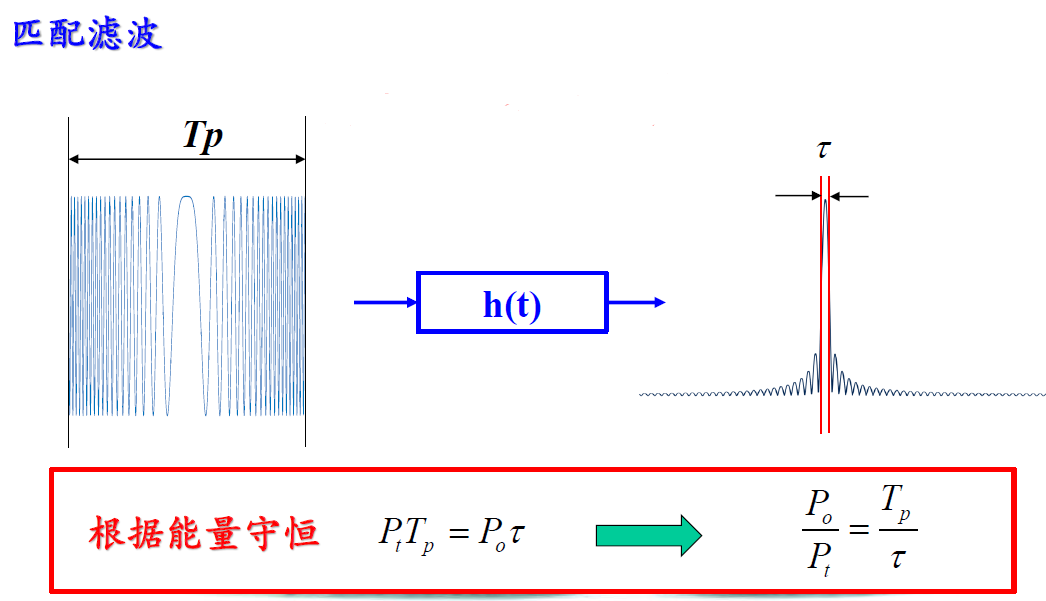由此可见，线性调频信号的匹配滤波器输出为sinc函数，其时宽为$\tau=\frac{1}{B}$（-4dB点）;
根据能量守恒，$\frac{P_0}{P_t}=\frac{T_p}{\tau}=BT_p=D$，输出脉冲峰值功率比输入脉冲峰值功率增大了D倍，也就是线性调频信号经过压缩处理后的接收信号幅度峰值是原来发射信号峰值的D的1/2次方。（D为时宽带宽积）

8.4 匹配滤波脉压输出性能分析

峰值旁瓣比：
峰值与最大的第一对旁瓣之比。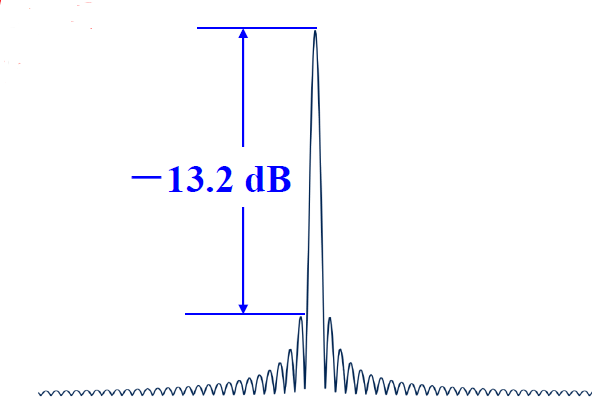高旁瓣影响：
强目标回波的旁瓣会埋没附近较小目标的主瓣，导致目标丢失。

解决方法：
为了提高分辨多目标的能力，必须采用旁瓣抑制或加权技术。

9.线性调频信号脉冲压缩的匹配滤波实现
对于线性调频信号
时域为：$s(t)=rect(\frac{t}{T})e^{j\pi K t^2}$
频谱为（驻定相位原理）：$S(f)=\frac{1}{\sqrt K}rect(\frac{f}{KT})e^{-j\pi \frac{f^2}{K}}e^{j\frac{\pi}{4}}$
9.1 时域匹配滤波

根据 $h(t)=s^*(-t)$可构造时域匹配滤波器为发射信号时间反褶再取共轭，再与发射信号进行线性卷积即可实现脉冲压缩。
$s0(t)=s(t)*s^*(-t)$

ht = conj(fliplr(st));
s = conv(st,ht);
9.2 频域匹配滤波
9.2.1 方法一

原理：

将发射信号时间反褶后取共轭得到h(t)，补零后计算FFT，再与信号补零后的FFT在频域相乘，最后IFFT。

ht = conj(fliplr(st));
Hf = fft(ht,Nfft);
Sf = fft(st,Nfft);
s = ifft(Hf.*Sf);

说明：
为什么需要补零？
因为匹配滤波需要计算线性卷积，但 (DFT) FFT 计算的是循环卷积，所以需要对信号进行补零直到长度超过线性卷积的长度。对于点数分别为N1与N2的两信号，它们线性卷积的长度为 N1+N2-1；若循环卷积长度为N，则有如下关系：
① N < N1 + N2 - 1 时，循环卷积是线性卷积长度为 N 的混叠；
② N = N1 + N2 - 1 时，循环卷积 = 线性卷积；
③ N > N1 + N2 - 1 时，循环卷积 = 线性卷积末尾补 N-(N1+N2-1) 个 0 （弃置区）。
弃置区位置
由于发射信号时间反褶后再补零，故最终得到IFFT结果后，弃置区位于信号前端。

9.2.2 方法二

原理：

将发射脉冲补零后进行FFT，再取共轭（无需反褶），与信号补零后的FFT在频域相乘，最后IFFT。

Hf = conj(fft(st,Nfft));
Sf = fft(st,Nfft);
s = ifft(Hf.*Sf);

说明：

方法二与方法一的关系？
根据傅里叶变换的性质，时域反褶+共轭 ↔ 频域共轭（方法一）；因此，也可先变换到频域再取共轭（方法二）。但由于它们补零时存在是否反褶的差别，故最终结果的弃置区也存在反褶。

弃置区位置
由于是直接在发射信号末尾补零，故最终得到IFFT结果后，弃置区位于信号末端。

9.2.3 方法三

直接在频域生成匹配滤波器:
$H(f)=\frac{1}{\sqrt K}rect(\frac{f}{KT})e^{j\pi \frac{f^2}{K}}e^{-j\frac{\pi}{4}}$
然后与信号的FFT在频域相乘，最后IFFT。

Hf = exp(1i*pi*f_h.^2/K);
Sf = fft(st,Nfft);
s = ifft(Hf.*Sf);
10.MATLAB仿真
10.1 线性调频信号的匹配滤波器输出

代码：

clear all;
close all;
clc;
%%******************* 线性调频与脉冲压缩 ********************%%
set(0,'defaultfigurecolor','w'); %设置MATLAB的图形窗口背景为白色
%% 线性调频信号参数设置
T = 1e-6;      %时宽
B = 200e6;     %带宽
Fs = 4*B;      %采样率

K = B/T;                          %调谐频率
N = round(T/(1/Fs) );             %采样点数，四舍五入
%% 产生线性调频信号
t = linspace( -T/2 ,T/2 ,N);
st = ( abs(t) < T/2 ) .* exp( 1j * pi * K * t.^2 );
phase = pi * K * t.^2;          %信号相位
f= K * t;                       %信号频率
%% 频谱
freq = linspace(-Fs/2,Fs/2,N);  %频域采样
Sf = fftshift( fft(st) );
%% 时域匹配滤波
% conj()函数用于计算复数的共轭值；
% fliplr()函数将数组从左到右翻转.
ht = conj( fliplr(st) );    %时域匹配滤波为发射信号时间反褶再取共轭：h(t)=s*(-t)
s1 = conv(st,ht);           %线性调频信号经过匹配滤波器后的输出(时域卷积)
N1 = N+N-1 ;                %线性卷积后信号长度变为 N1+N2-1
t1 = linspace( -T/2 , T/2 , N1);
%% 频域匹配滤波1 (复制发射脉冲进行时间反褶并取共轭，计算补零DFT)
N2 = 2*N;                    %循环卷积长度 （N2应当≥N+N-1，其中弃置区位于长度大于N+N-1的部分）
t2 = linspace( -T/2 , T/2 , N2);
Hf1 = fftshift(fft(ht,N2));  %频域匹配滤波器
Sf2 = fftshift(fft(st,N2));  %频域信号
S2 = Sf2 .* Hf1;             %频域乘积
s2 = ifft(S2);
%% 频域匹配滤波2（复制发射脉冲补零后计算FFT，再取共轭）
Hf2 = fftshift(conj(fft(st,N2)));
S3  = Sf2.* Hf2;
s3  = fftshift(ifft(S3));
%% 频域匹配滤波3（直接生成频域滤波器）
f_h = (-N2/2:N2/2-1)*Fs/N2;
Hf3 = exp(1i*pi*f_h.^2/K);
S4 = Sf2.*Hf3;
s4 = ifft(S4);
%% 绘图
% 时域
figure,plot( t*1e6, real(st) ),xlabel('t /us'),ylabel('幅度'),title('Chirp信号 实部');
figure,plot( t*1e6, imag(st) ),xlabel('t /us'),ylabel('幅度'),title('Chirp信号 虚部');
figure,plot( t*1e6, f*1e-6 ),xlabel('t /us'),ylabel('频率 /MHz'),title('Chirp信号 频率');
figure,plot( t*1e6, phase ),xlabel('t /us'),ylabel('相位 /rad'),title('Chirp信号 相位');
% 频域
figure,plot( freq*1e-6,abs(Sf) ),xlabel('f /MHz'),ylabel('幅度谱'),title('Chirp信号 幅度谱');
figure,plot( freq*1e-6,-pi*freq.^2/K+pi/4 ),xlabel('f /MHz'),ylabel('相位谱'),title('Chirp信号 相位谱');
% 时域匹配滤波
figure,plot( t1*1e6 , abs(s1) ),xlabel('t /us'),ylabel('幅度谱'),title('时域匹配滤波');
% 频域匹配滤波1
figure,plot( t2*1e6 , abs(s2) ),xlabel('t /us'),ylabel('幅度谱'),title('频域匹配滤波1');
% 频域匹配滤波2
figure,plot( t2*1e6 , abs(s3) ),xlabel('t /us'),ylabel('幅度谱'),title('频域匹配滤波2');
% 频域匹配滤波3
figure,plot( t2*1e6 , abs(s4) ),xlabel('t /us'),ylabel('幅度谱'),title('频域匹配滤波3');


结果：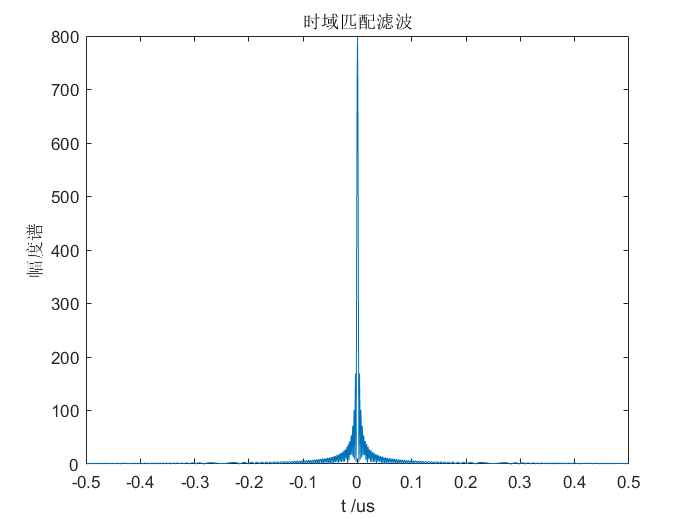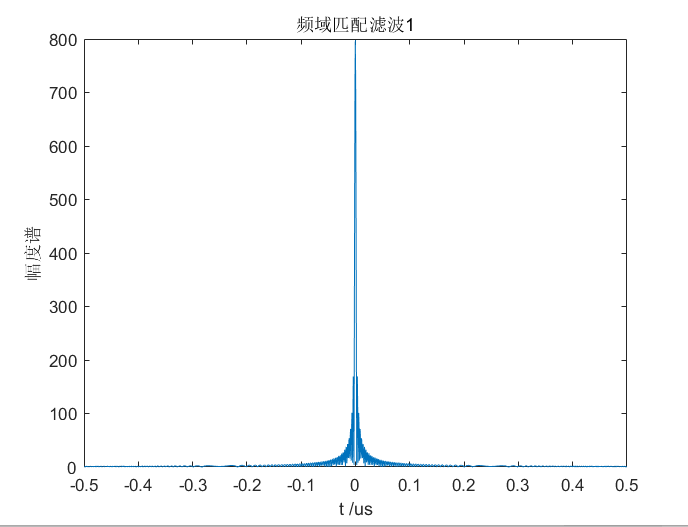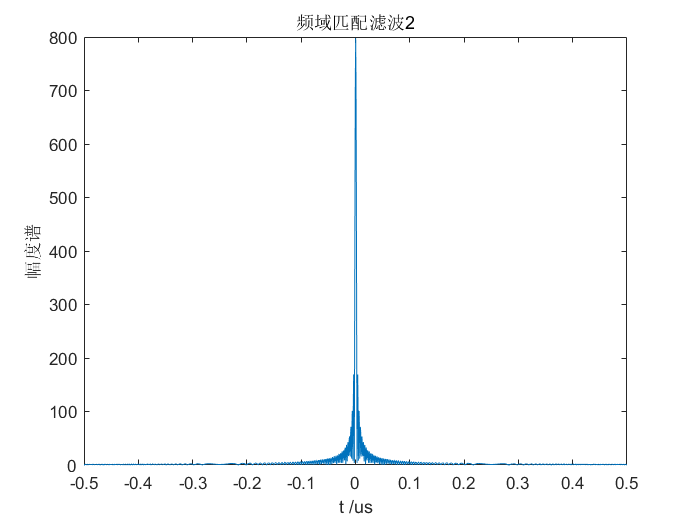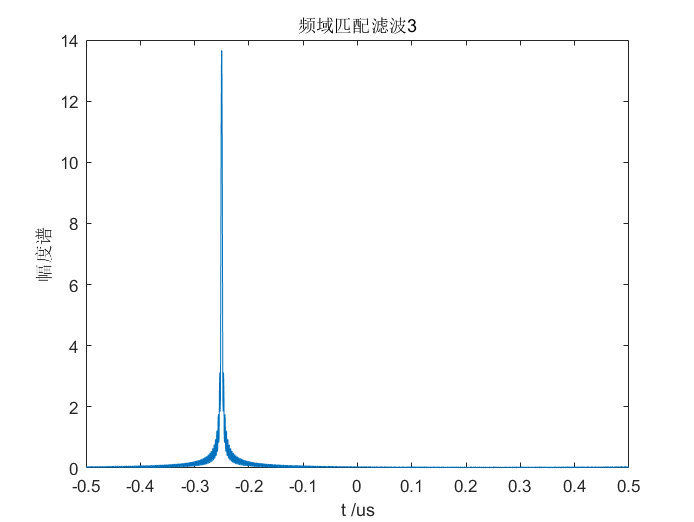10.2 利用匹配滤波器对回波信号进行脉冲压缩来测距

代码：

%%------------------------------------------------------------------------------------------------------------%%
%%说明：使用匹配滤波器进行脉冲压缩，matched_filter函数的输出包括未压缩和压缩的信号图形，以及在脉冲压缩中使用的线性调频信号%%
%%------------------------------------------------------------------------------------------------------------%%
function [y] =matched_filter(nscat,taup,b,rrec,scat_range,scat_rcs,winid)
%********* y: 归一化的压缩输出 **************%
%***** nscat: 目标个数 *********************%
%****** taup: 信号时宽，单位为s *************%
%********* b: 信号带宽，单位为Hz ************%
%****** rrec: 接收窗的大小，单位为m *********%
% scat_range: 目标的相对距离向量，单位为m ****%
%** scat_rcs: 目标的雷达截面积向量，单位为m^2 %
%***** winid: 决定了选用什么样的滤波器形式 ***%

%%%%% 时宽带宽积--time bandwidth product %%%%%
T_B_product=b*taup;
if(T_B_product<5)
fprintf('**********Time Bandwidth product is TOO SMALL**********')
fprintf('\nChange b and or taup');
return
end

c=3e8;           %光速--speed of light
n=fix(5*taup*b); %采样点数--number of samples;函数fix()：向零方向取整
%根据奈奎斯特采样定理及频率分辨率△f=fs/N的要求可以确定采样点数N的取值

%%%%% 初始化输入输出--initialize input,output %%%%%
x(nscat,1:n)=0;
y(1:n)=0;
%%%%% 确定合适的接收窗--determine proper window %%%%%
if(winid==0)              %不加窗
win(1:n)=1;
win=win';
else
if(winid==1)          %汉明窗
win=hamming(n);
else
if(winid==2)      %带参数pi的凯泽窗
win=kaiser(n,pi);
else
if(winid==3)  %旁瓣为-60dB的切比雪夫窗
win=chebwin(n,60);
end
end
end
end
%%%%%% 确保目标在接收窗内--check to ensure that scatters are within receive window %%%%%
index=find(scat_range>rrec);
if(index~=0)
fprintf('Error.Receive window is too large or scatters fall outside window');
return
end
%%%%% 计算采样间隔--calculate sampling interval %%%%%
t=linspace(-taup/2,taup/2,n);
LFM=exp(1i*pi*(b/taup).*t.^2); %载频为0的线性调频信号

figure(1);
subplot(2,1,1);
plot(t,real(LFM));
xlabel('时间/s');
ylabel('线性调频信号的实部');
grid on;
subplot(2,1,2);
sampling_interval=taup/n;
freqlimit=1/sampling_interval;            %采样频率Fs=1/Ts
freq=linspace(-freqlimit/2,freqlimit/2,n);
plot(freq,fftshift(abs(fft(LFM))));
xlabel(' 频率/Hz');
ylabel('线性调频信号的幅度谱');
grid on;

for j=1:1:nscat
range=scat_range(j);
x(j,:)=scat_rcs(j).*exp(1i*pi*(b/taup).*(t+2*range/c).^2); %第j个目标的回波信号
y=x(j,:)+y;                                                %实现对nscat个目标回波信号的叠加
end

figure(2);
plot(t,real(y),'m');
xlabel('相对延迟/s');
ylabel('未压缩回波');
grid on;

out=xcorr(LFM,y);       %xcorr(x,y):互相关函数
out=out./n;
s=taup*c/2;
Npoints=ceil(rrec*n/s); %ceil():朝正无穷方向取整
dist=linspace(0,rrec,Npoints);

figure(3);
plot(dist,abs(out(n:n+Npoints-1)),'k');
xlabel('目标相对距离');
ylabel('压缩回波');
grid on;
end


结果：

2个目标的回波信号的匹配滤波

clear all;
close all;
clc;
%%调用matched_filter函数，观察结果，这两个目标回波是重叠的，不能被分辨，但是脉冲压缩后，这两个脉冲是完全分开的，即可以被分辨出来
nscat=2;             %两个目标
taup=10e-6;          %10us信号时宽
b=50.0e6;            %50MHz脉宽
rrec=50;             %50m的接收窗
scat_range=[15 25];  %目标的相对距离分别为15m、25米
scat_rcs=[1 2];      %目标的雷达截面积分别是1m^2和2m^2
winid=0;             %不加窗
[y]=matched_filter(nscat,taup,b,rrec,scat_range,scat_rcs,winid);3个目标的回波信号的匹配滤波

clear all;
close all;
clc;
%%%%%%%%%% 调用matched_filter函数观测3个目标相对距离为[10 75 120]m的结果 %%%%%%%%%%
nscat=3;                 %3个目标
taup=5e-6;               %5us非压缩脉冲
b=100e6;                 %100MHz脉宽
rrec=200;                %200m的处理窗
scat_range=[10 75 120];  %目标相对雷达的距离分别为10m、75m及120m
scat_rcs=[1 2 1];        %目标的雷达截面积分别是1m^2、2m^2及1m^2
winid=2;                 %凯泽窗
[y]=matched_filter(nscat,taup,b,rrec,scat_range,scat_rcs,winid);展开全文信号处理 matlab 卷积
• 我自己整理的关于匹配滤波器的资料，有需要的朋友可以下
• 从频域直观理解匹配滤波器的效果(2) 匹配滤波器的冲激响应(3) 匹配滤波器的输出信噪比(4) 匹配滤波器的时间适应性(5) 匹配滤波器与自相关(6) 匹配滤波器进行频域滤波 参考 通信之道——从微积分到5G 匹配滤波器...
文章目录(1) 匹配滤波器的公式推导与解释1.使用匹配滤波器的目的2.推导匹配滤波器的公式3.从频域直观理解匹配滤波器的效果(2) 匹配滤波器的冲激响应(3) 匹配滤波器的输出信噪比(4) 匹配滤波器的时间适应性(5) 匹配滤波器与自相关(6) 匹配滤波器进行频域滤波

参考

通信之道——从微积分到5G
匹配滤波器及其在雷达信号处理中的应用
随机过程概论（PPT） 第四章
线性调频信号

(1) 匹配滤波器的公式推导与解释
解释实际上是要分三步进行：首先要说明要用匹配滤波器干了一个什么事，其次要通过匹配滤波器要做的事说明为什么匹配滤波器的公式是这个形式，最后又要从直观的角度来说明匹配滤波器的这个公式为什么能让它做成这个事情。
1.使用匹配滤波器的目的

当有用信号通过信道传输后，混杂有许多高斯白噪声，信号被噪声淹没。通过匹配滤波器提高信噪比，使有用信号在时域上更加明显，更易被检测到。

2.推导匹配滤波器的公式

推导公式从匹配滤波器要实现的功能出发：提高信噪比。
一个实信号 $s(t)$ 通过 AWGN 信道，得到 $r(t) = s(t) + n(t)$。其中 $n(t)$ 是高斯白噪声。同时已知的高斯白噪声的相关函数 $R(\tau) = \displaystyle\frac{N_0}{2}\delta(\tau)$
让被污染的信号通过一个时域冲激响应为 $h(t)$ 的系统并在 $t_0$ 时刻采样可以得到
\begin{aligned} y(t_0) &= \int_{-\infin}^{\infin}r(\tau)h(t_0-\tau)d\tau\\ &=\int_{-\infin}^{\infin}s(\tau)h(t_0-\tau)d\tau + \int_{-\infin}^{\infin}n(t)h(t_0-t)dt\\ &=y_s(t_0) + y_n(t_0) \end{aligned}
很容易发现，$y_s(t_0)$ 是有用信号，$y_n(t_0)$ 是噪声信号，由此定义信噪比$SNR = \displaystyle\frac{y_s^2(t_0)}{E[y_n^2(t_0)]}$
同时可以推导出 $E[y_n^2(t_0)]$ 的表达式
\begin{aligned} E[y_n^2(t_0)] &= E[\int_{-\infin}^{\infin}n(\tau)h(t_0-\tau)d\tau\int_{-\infin}^{\infin}n(t)h(t_0-t)dt]\\ &=\int_{-\infin}^{\infin}\int_{-\infin}^{\infin}\frac{N_0}{2}\delta(t-\tau)h(t_0-\tau)h(t_0-t) d\tau dt\\ &=\frac{N_0}{2}\int_{-\infin}^{\infin}h^2(t_0-\tau) d\tau \end{aligned}
回代并求出最大值
\begin{aligned} SNR&=\displaystyle\frac{2[\displaystyle\int_{-\infin}^{\infin}s(\tau)h(t_0-\tau)d\tau]^2}{N_0\displaystyle\int_{-\infin}^{\infin}h^2(t_0-\tau)d\tau}\le \displaystyle\frac{2\displaystyle\int_{-\infin}^{\infin}s^2(\tau)d\tau\displaystyle\int _{-\infin}^{\infin}h^2(t_0-\tau)d\tau}{N_0\displaystyle\int_{-\infin}^{\infin}h^2(t_0-\tau)d\tau}\\ &=\displaystyle\frac{2}{N_0}\displaystyle\int_{-\infin}^{\infin}s^2(\tau)d\tau \end{aligned}
上面的式子在放缩的过程中使用了柯西不等式，所以想要达到最大值就需要根据柯西不等式等号成立的条件得到 $h(t_0-\tau) = s(\tau)\Rightarrow h(t) = ks(t_0-t)$

3.从频域直观理解匹配滤波器的效果

由时域表达式可以推出频域表达式 $H(\omega) = kS^*(\omega)e^{-j\omega t_0}$，可以发现有 $|H(\omega)| = k|S(\omega)|$，也就是说系统的增益和信号的幅度谱是一样的。
由于白噪声的频谱是常数，就是说在哪里都一样，所以系统在信号幅度谱更大的地方（含有的有用信号会更多）使用更大的增益就可以让有用信号在总的信号中增长的更多，也就是说提高了信噪比。

(2) 匹配滤波器的冲激响应

由之前的推证可以知道，冲激响应的时域表达式为 $h(t) = ks(t_0-t)$。

注意 $h(t)$ 与 $t_0$ 值选取需要遵循的准则：
因果关系
$h(t) = \begin{cases} s(t_0-t),t\ge 0\\ 0,t<0 \end{cases}$
为了使输入信号的全部都能对输出信号有所贡献，采样时刻 $t_0$ 应满足 $s(t)=0,t>t_0$。比如信号产生的时间是 $[0,10\mu s]$，采样时刻 $t_0$ 就至少是 $10\mu s$。

这里假定采样时刻 T = 12$\mu$s，信号带宽 B = 20MHz，信号时宽 TimeWidth = 10$\mu$s，信号的采样频率 $F_s$ = 50MHz，起始频率为 0Hz。

matlab 代码
%% 产生线性调频波
TimeWidth = 10e-6;      %脉冲持续时间10us
T = 8e-6;               %T在8us
BandWidth = 20e6;       %线性调频信号的频带宽度20MHz
Fs = 50e6;              %采样频率，注意需要满足奈奎斯特频率
sample_dot_num = round(TimeWidth * Fs);%表示采样点的个数

f0 = 0;%初始频率
f1 = f0 + BandWidth;%终止频率
t=0:1/Fs:TimeWidth;%根据结束时间生成时间序列
signal = chirp_signal(t,f0,f1);
plot(t*1e6,signal,'LineWidth',2);
%% 匹配滤波器的脉冲响应
%这里 k 假定取 1
h = signal(end:-1:1);
t = -t(end:-1:1) + T;
plot(t*1e6,h,'LineWidth',2);


仿真结果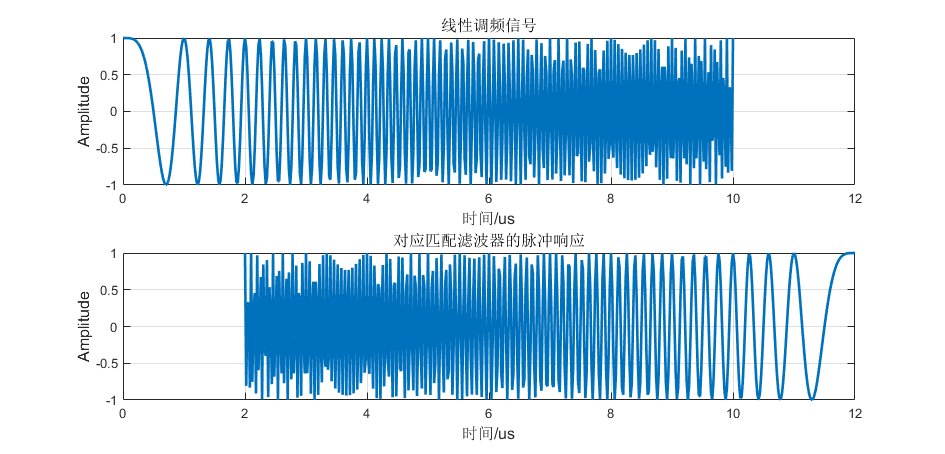(3) 匹配滤波器的输出信噪比

匹配滤波器的输出功率信噪比只取决于输入信号的能量和白噪声功率谱密度而与输入信号形状和噪声分布规律无关。

这里在仿真时均假定 snr=0，并且通过增添频率移动量保证整体能量基本相等，均为 $125.5$ 左右。

matlab 代码：
%% 产生线性调频信号
TimeWidth = 10e-6;      %脉冲持续时间10us
BandWidth = 20e6;       %线性调频信号的频带宽度20MHz
Fs = 50e6;Ts = 1/Fs;    %采样频率，注意需要满足奈奎斯特频率
N = fix(TimeWidth/Ts);
f0 = 0;%初始频率
f1 = f0 + BandWidth;%终止频率

%设定频率偏移量
f_shifta = 5e6;
f_shiftb = 10e6;
t=0:1/Fs:TimeWidth;%根据结束时间生成时间序列
%采用不同的频率偏移量生成两个能量（几乎）相同的信号
signal1 = chirp_signal(t,f0+f_shifta,f1);
signal2 = chirp_signal(t,f0+f_shiftb,f1);
plot(t*1e6,signal1);
plot(t*1e6,signal2);
%% 加入噪声后
%加入噪声
snr = 0;
y1 = awgn(signal1, snr, 'measured');
y2 = awgn(signal2, snr, 'measured');
plot(t*1e6,y1);
plot(t*1e6,y2);
% 经过匹配滤波器
mf=fliplr(signal1);     %输入序列取反序（匹配滤波器的脉冲响应）
sr_noise_mf=conv(mf,y1); %有噪声回波信号匹配滤波（使用脉冲响应和输入信号进行卷积）
plot((0:length(sr_noise_mf)-1)/Fs*1e6,20*log10(abs(sr_noise_mf)))
mf=fliplr(signal2);     %输入序列的复共轭（匹配滤波器的脉冲响应）
sr_noise_mf=conv(mf,y2); %有噪声回波信号匹配滤波（使用脉冲响应和输入信号进行卷积）
plot((0:length(sr_noise_mf)-1)/Fs*1e6,20*log10(abs(sr_noise_mf)))


仿真结果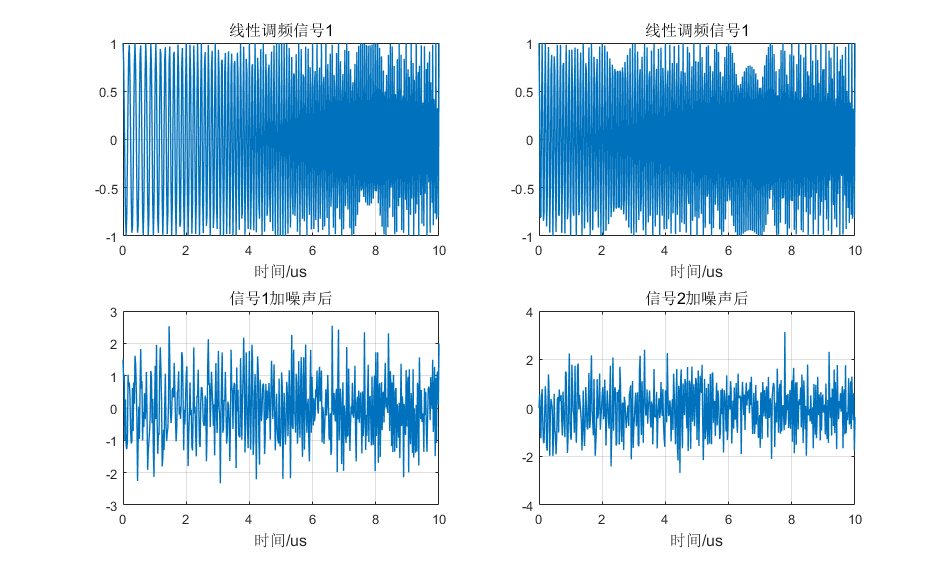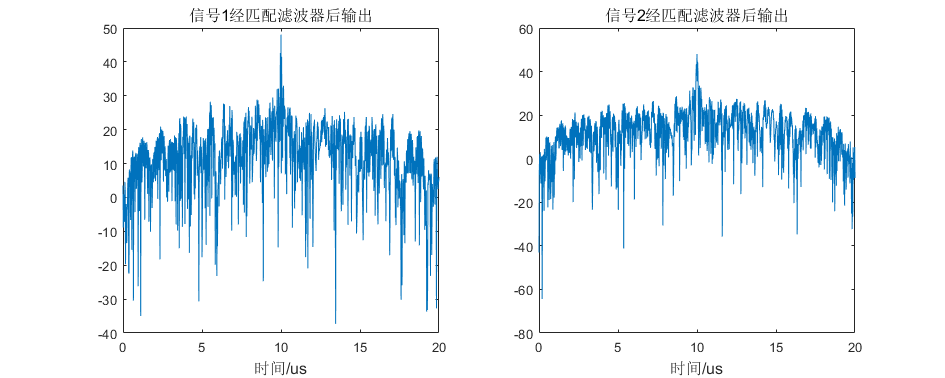可以看到在信号能量基本相等的情况下，通过匹配滤波器后的信噪比最大的位置基本相同。

(4) 匹配滤波器的时间适应性

匹配滤波器对振幅和时延参量不同的信号具有适应性，具体来说就是与信号 $s(t)$ 相匹配的滤波器的系统函数 $H(\omega)$ ，对于信号 $s_1(t) = As(t-\tau)$ 来说也是匹配的，只不过最大输出功率信噪比出现的时刻延迟了 $\tau$。
matlab 代码%% 产生线性调频信号
TimeWidth = 10e-6;      %脉冲持续时间10us
TimeEmpty = 20e-6;
BandWidth = 20e6;       %线性调频信号的频带宽度20MHz
Fs = 50e6;Ts = 1/Fs;    %采样频率，注意需要满足奈奎斯特频率
N = fix(TimeWidth/Ts);

f0 = 0;%初始频率
f1 = f0 + BandWidth;%终止频率
t=0:1/Fs:TimeWidth;%根据结束时间生成时间序列
signal = chirp_signal(t,f0,f1);
t_total = linspace(0,2*(TimeEmpty+TimeWidth),2*(TimeEmpty+TimeWidth)/Ts);
signal_total = [signal(1:end-1),zeros(1,fix(TimeEmpty/Ts)),signal(1:end-1),zeros(1,fix(TimeEmpty/Ts))];
plot(t_total*1e6,signal_total,'LineWidth',2);
%% 加入噪声后
%加入噪声
snr = 0;
signal_with_noise = awgn(signal_total, snr, 'measured');
plot(t_total*1e6,signal_with_noise,'LineWidth',2);
%% 经过匹配滤波器后(信号波形通过与其对应的匹配滤波器)
%这里 k 假定取 1
h=zeros(1,N);
t1 = fix(TimeWidth/Ts);
for tt=0:N-1
h(tt+1)=signal_total(t1-tt);%匹配滤波器
end
% 经过匹配滤波器
sr_noise_mf=conv(h,signal_with_noise); %有噪声回波信号匹配滤波（使用脉冲响应和输入信号进行卷积）
plot((0:length(sr_noise_mf)-1)/Fs*1e6,20*log10(abs(sr_noise_mf)),'LineWidth',2);


仿真结果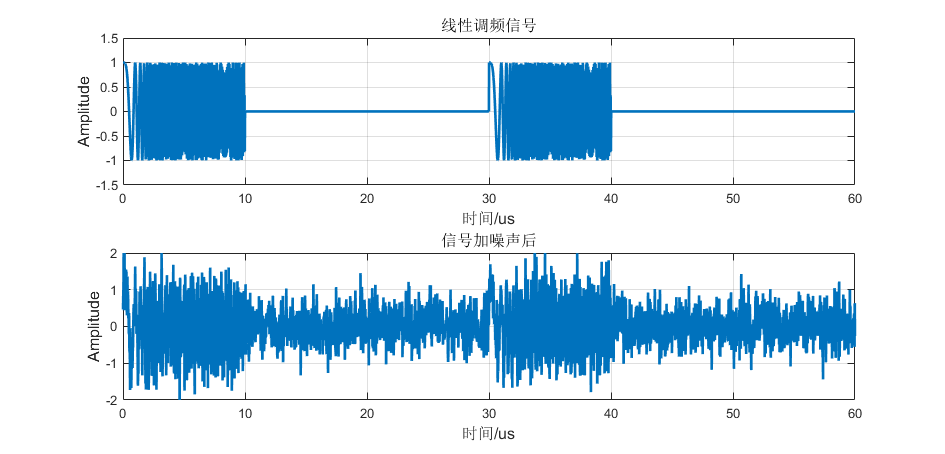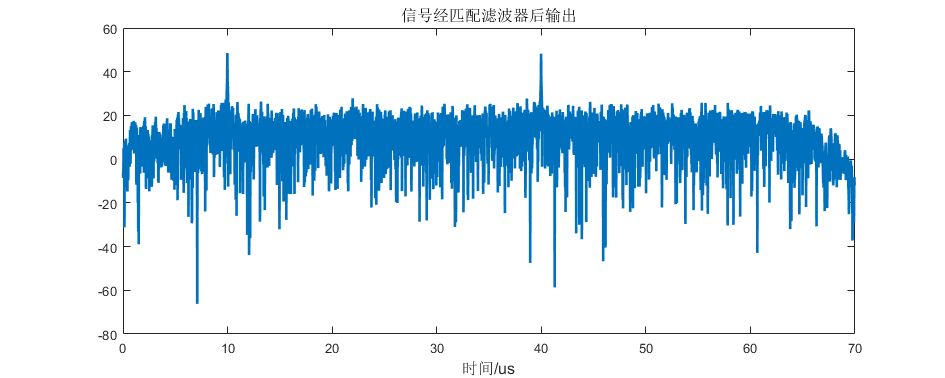可以看出来，对于有一个时延的信号，使用相同的匹配滤波器会导致输出在信噪比最大的地方也有一个响应的时延。

(5) 匹配滤波器与自相关

matlab代码%% 产生线性调频信号
TimeWidth = 10e-6;      %脉冲持续时间10us
BandWidth = 20e6;       %线性调频信号的频带宽度20MHz
Fs = 50e6;Ts = 1/Fs;    %采样频率，注意需要满足奈奎斯特频率
N = fix(TimeWidth/Ts);
f0 = 0;%初始频率
f1 = f0 + BandWidth;%终止频率
t=0:1/Fs:TimeWidth;%根据结束时间生成时间序列
signal = chirp_signal(t,f0,f1);
signal = awgn(signal,0,'measured');%这里添加白噪声
plot(t*1e6,signal,'LineWidth',2);
%% 匹配滤波
%计算匹配滤波器的冲激响应函数
h = zeros(1,N);
t1 = fix(TimeWidth/Ts);
for tt=0:N-1
h(tt+1) = signal(t1-tt);%匹配滤波器
end
signal_match = abs(conv(h,signal));
t_match = (0:length(signal_match)-1)/Fs;
plot(t_match*1e6,signal_match,'LineWidth',2);
%% 求自相关函数
signal_autocorrelation = abs(xcorr(signal));
t_autocorrelation = (0:length(signal_autocorrelation)-1)/Fs;
plot(t_autocorrelation*1e6,signal_autocorrelation,'LineWidth',2);


仿真结果：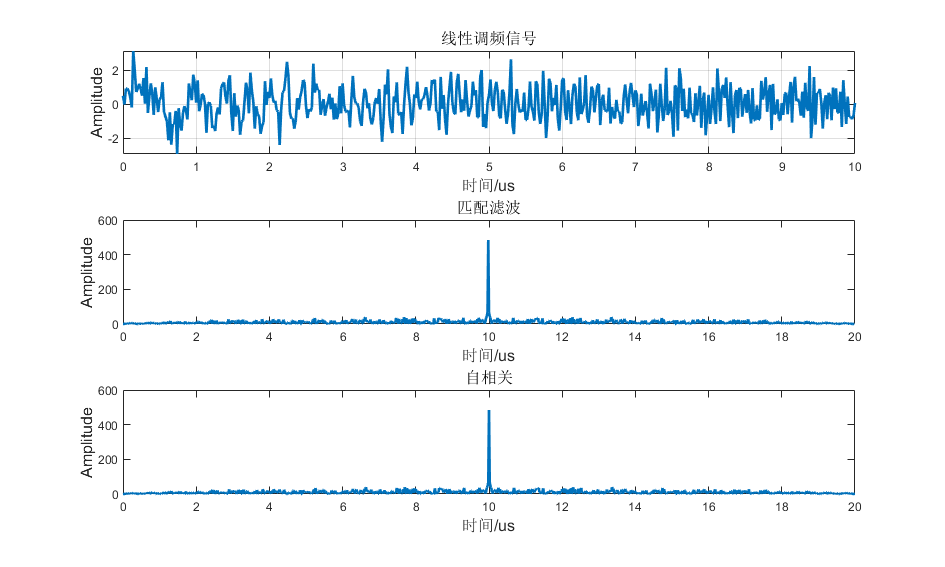从波形上来看两者是完全相同的，但是一定要注意前提条件。在 $t=t_0$ 并且噪声 $n(t)$ 为零均值白噪声的条件下匹配滤波器与相关器的输出是相等的。

(6) 匹配滤波器进行频域滤波

matlab 代码
%% 产生线性调频信号
TimeWidth = 10e-6;      %脉冲持续时间10us
BandWidth = 20e6;       %线性调频信号的频带宽度20MHz
Fs = 50e6;Ts = 1/Fs;    %采样频率，注意需要满足奈奎斯特频率
N = fix(TimeWidth/Ts);
f0 = 0;%初始频率
f1 = f0 + BandWidth;%终止频率
t=0:1/Fs:TimeWidth;%根据结束时间生成时间序列
signal = chirp_signal(t,f0,f1);

snr = 0;
signal_with_noise = awgn(signal, snr, 'measured');  %回波信号实际上就是给原信号增加了噪声
plot(t*1e6,signal_with_noise,'LineWidth',2);

freq=linspace(0,Fs,N);
plot(freq*1e-6,fftshift(abs(fft(signal_with_noise(1:end-1)))),'LineWidth',2);

%% 进行匹配滤波
%计算匹配滤波器的冲激响应函数
h = zeros(1,N);
t1 = fix(TimeWidth/Ts);
for tt=0:N-1
h(tt+1) = signal(t1-tt);%匹配滤波器
end
subplot(311)
plot(freq*1e-6,abs(fftshift(fft(h)).* fftshift(fft(signal_with_noise(1:end-1)))),'LineWidth',2);

%% 求相关
temp = abs(ifft(fftshift(fft(h)).* fftshift(fft(signal_with_noise(1:end-1)))));
temp = [temp,temp(end:-1:1)];
plot((0:length(temp)-1)*Ts*1e6,temp,'LineWidth',2);
%% 求匹配
subplot(313)
temp = abs(conv(h,signal_with_noise(1:end-1)));
plot((0:length(temp)-1)*Ts*1e6,temp,'LineWidth',2);


仿真结果：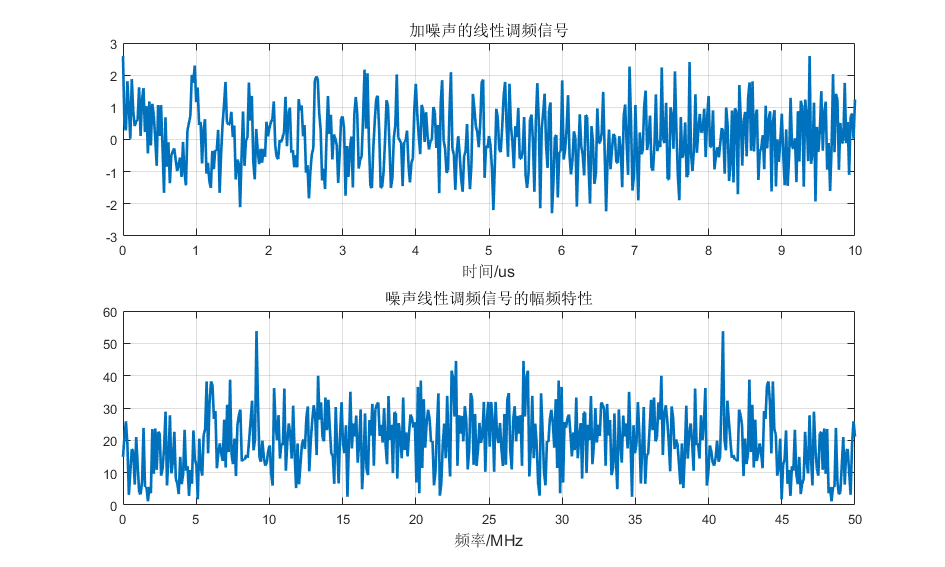对淹没在 0dB 高斯白噪声中的信号直接进行 FFT，可以看到在频域并不能很好的分出信号。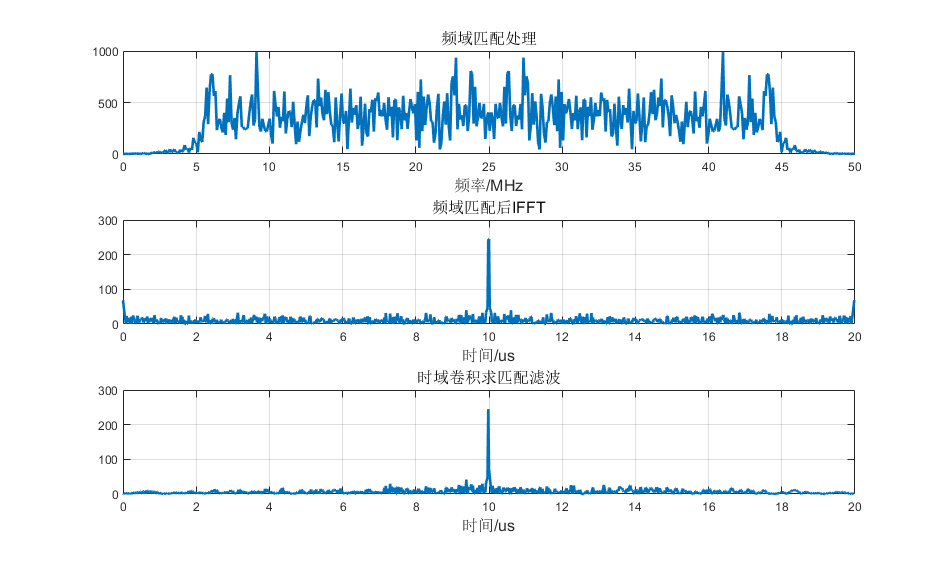频域乘积：对加噪信号和匹配滤波器脉冲响应分别进行 FFT 变换，得到两组频谱后点乘然后进行 IFFT 变换即可得出滤波结果。
从时域卷积：让加噪信号和匹配滤波器进行卷积，直接得到结果，可以看得这两种操作使结果 基本相同。


展开全文• WCDMA中规定了小区搜索的时隙同步过程采用匹配滤波器的方法实现，本论文主要研究匹配滤波器原理及FPGA实现结构。
• 匹配滤波器入门简介，介绍了关于匹配滤波器的基本知识，匹配滤波原理以及匹配方法
• 匹配滤波器：接收输出信噪比最大
写在前面：本文截屏自西安电子科技大学曹丽娜
视频学习地址：https://www.bilibili.com/video/BV1B7411o7Jo?p=34
仅供个人学习和构建知识体系所用。
匹配滤波器
本章研究目的：在高斯白噪声干扰下，如何检测信号，使接收性能最佳。这里的最佳指的是在某种准则下的最佳。
内容主线

主题
内容

最佳接收准则
似然准则

最佳接收器件
相关器，匹配滤波器

确知信号最佳接收
结构，误码性能

随相信号最佳接收
结构，误码性能

匹配滤波器：在最大化信号的同时，尽量减小噪声影响的线性滤波器设计方案。
设计准则：设计匹配滤波器的传输函数$H(f)或者h(t)$,使得输出信噪比在抽样时刻$T_0$有最大值。
输出信噪比ro定义为输出信号瞬时功率和输出噪声平均功率之比。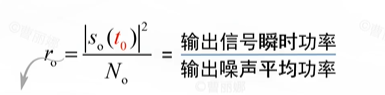传输函数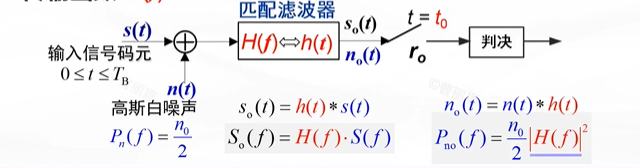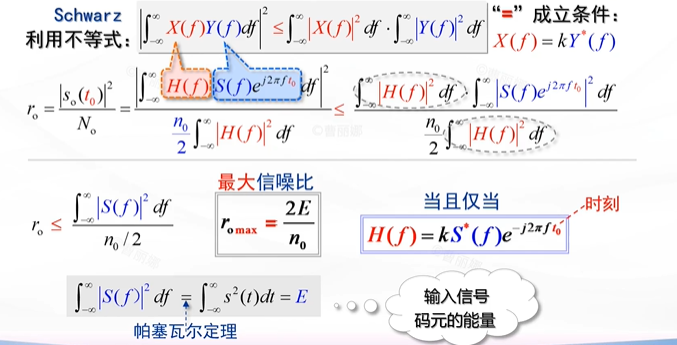下面对传输函数进行物理解释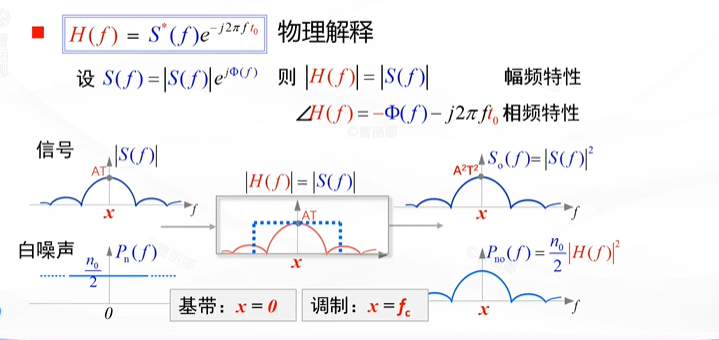下面从时域角度看匹配滤波器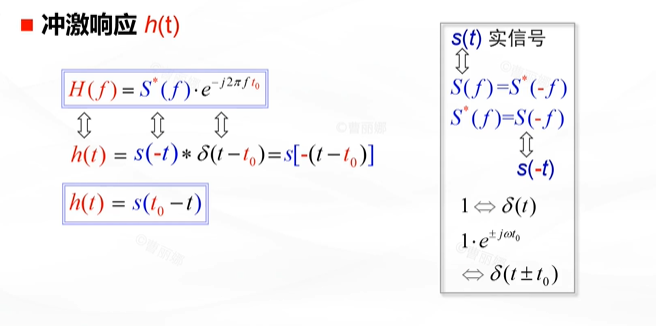含义是：h(t)是输入信号s(t)的镜像s(-t)及右移$t_0$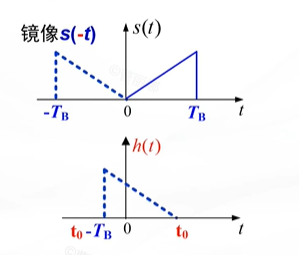接下来的问题是：时延$t_0$如何确定?
对于物理可实现的匹配滤波器，也就是因果系统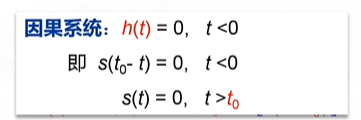这表示s(t）必须在t0之前结束，换言之，t0需要大于等于s(t)的持续时间TB，一般取$t_0=T_B$,这意味着每个码元的终止时刻即为最佳抽样时刻t0。
下面看输出信号$S_o(t)$
输出信号等于输入信号和匹配滤波器冲激函数的卷积，我们发现输出信号等于输入信号s(t)的自相关函数，换言之，匹配滤波器相当于相关器。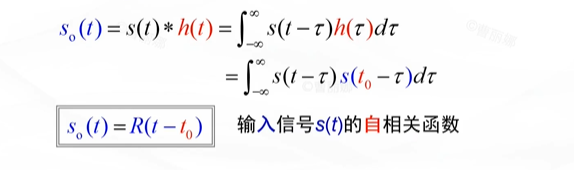在$t=t_0$时，输出信号有最大值，即输入信号码元的能量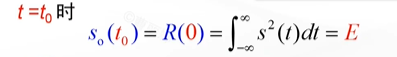下面看一道例题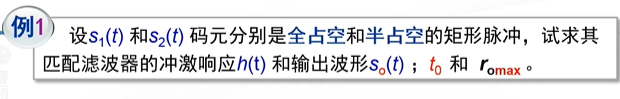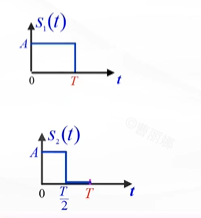解答：我们已经知道t0取输入码元的终止时刻，是上图中的T，而不是T/2.根据$h(t)=s(t_0-t)$
这是说对原信号s(t)反转，并延时（右移）t0【补充：这里是左移还是右移，需要对时域信号反转，平移性质有较好的理解：s(t)反转之后变成s(-t),然后朝哪边移动，是对t而言，s(-(t-t0))=s(t0-t)，按照左加右减，这是右移，即延时】
可以得到h1(t)和h2(t)，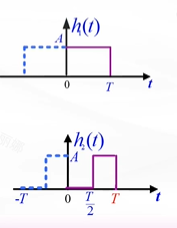可以求得输出信号：两个等宽方波信号卷积得到三角波信号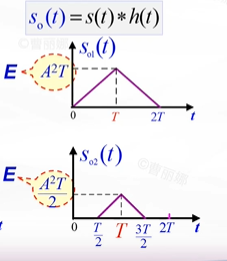在T时刻可以求得最大输出信噪比
根据公式$r_o max=\frac{2E}{n_0}$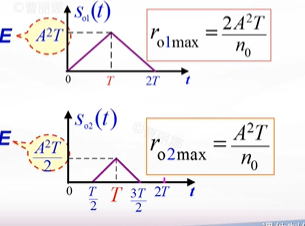最大输入信噪比与输入信号有效作用时间和输入信号的幅值有关，有效作用时间越长，效果越好，而和输入信号的波形无关。
下面看例题2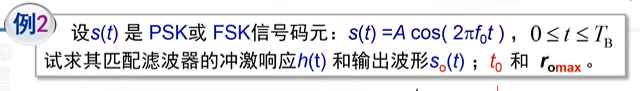解题技巧：两个矩形波包络的卷积，其输出信号的包络是三角波，所以可以看到输出信号的波形如下图所示。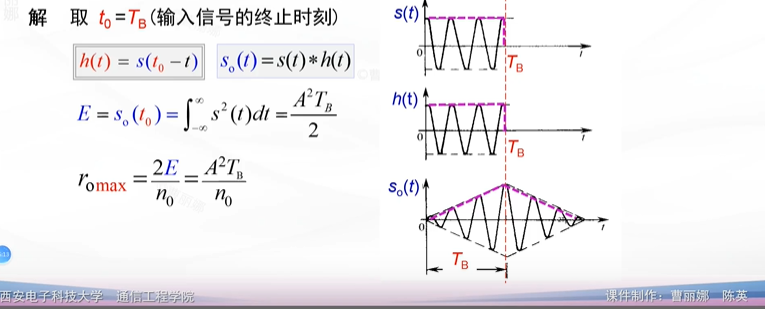匹配滤波器小结
匹配滤波器是一种在抽样时刻t0获得最大输出信噪比的最佳线性滤波器。
匹配滤波器的应用
适用于任何一种数字信号波形的接收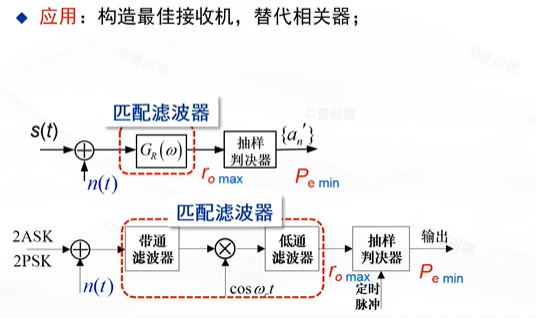思考题：匹配滤波器是否能够用于模拟信号的接收？


展开全文• 数字匹配滤l丧器具有很强的信号处理能力，是通信系统中常用的一种部件。文中介绍可变长度的通用敷字匹配滤波器原理与设计
• 关于匹配滤波器原理以及其matlab实现方式的详细过程 匹配滤波器的简单实现
• 目的: 提高信号检测能力和测量参量精度在所有线性滤波器中，匹配滤波器能给出最大峰值信噪比，它只取决于输入信号能量和白噪声功率谱密度，而 与输入信号形状和噪声分布规律无关。当白噪声功率谱密度一定时，为了...


请点击收听曲老师的音频：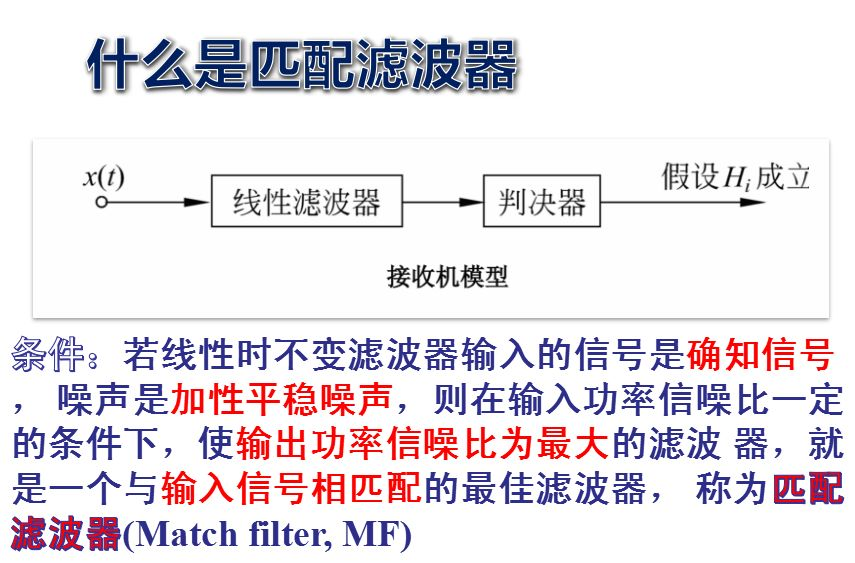匹配滤波器是一种最佳线性滤波器，准则的要求：①物理可实现；②唯一解答；③能求解的数学表达式。目的:  提高信号检测能力和测量参量精度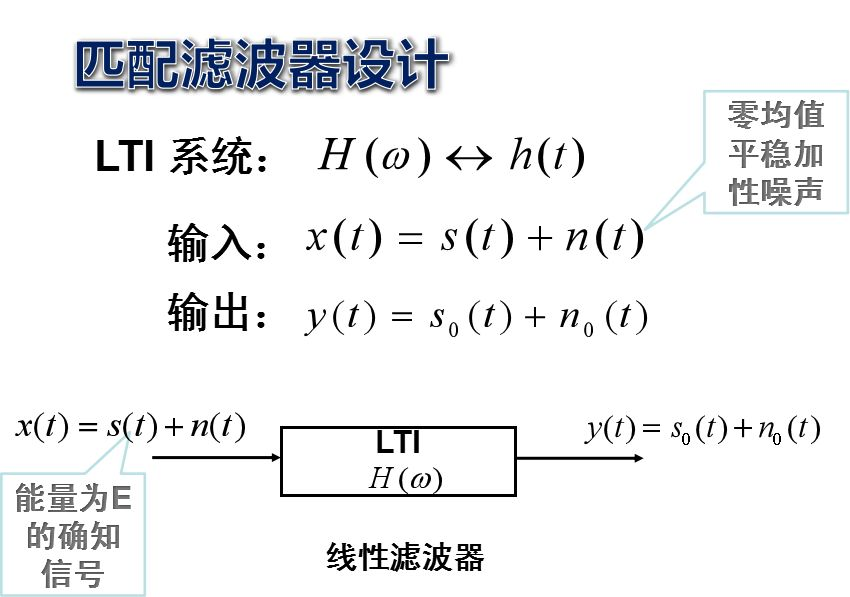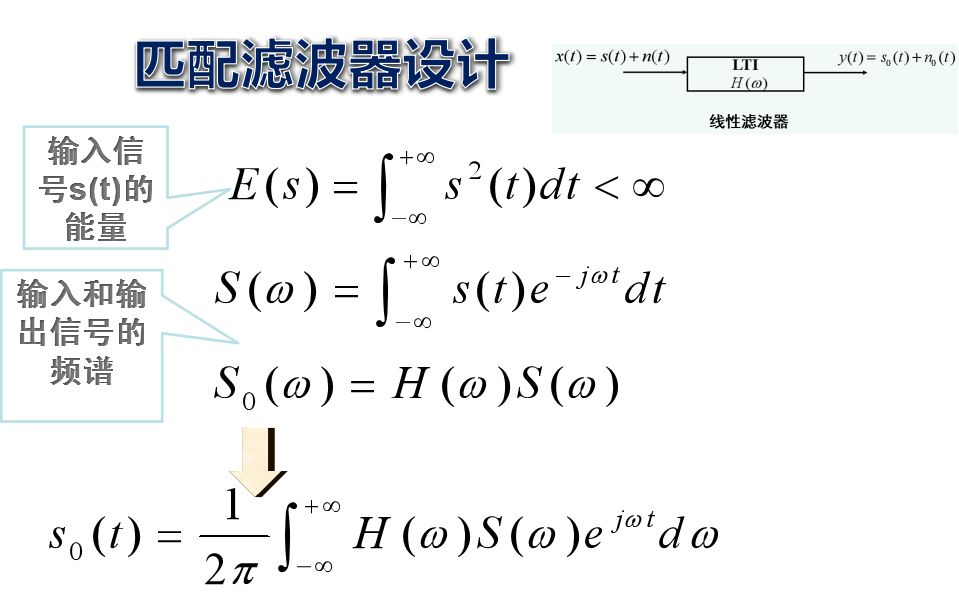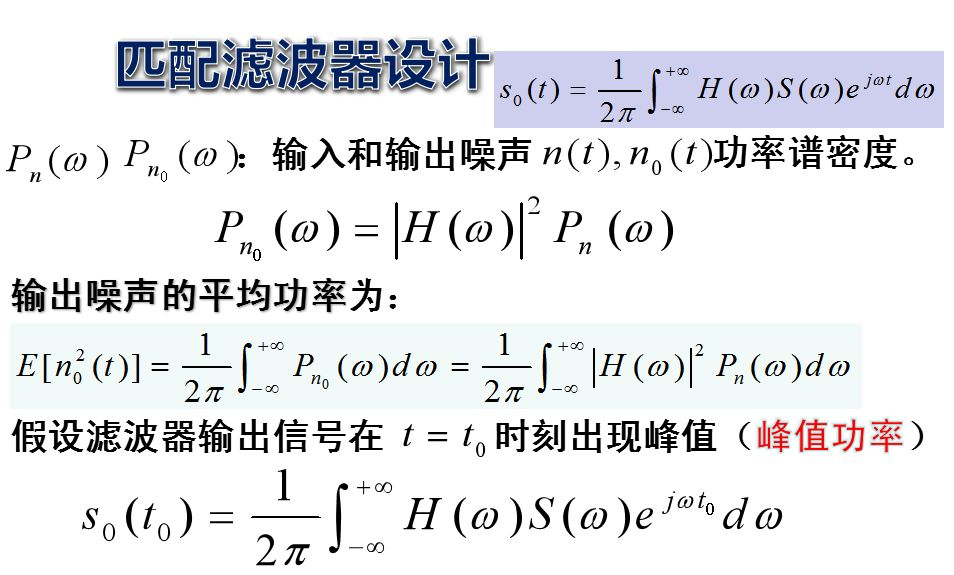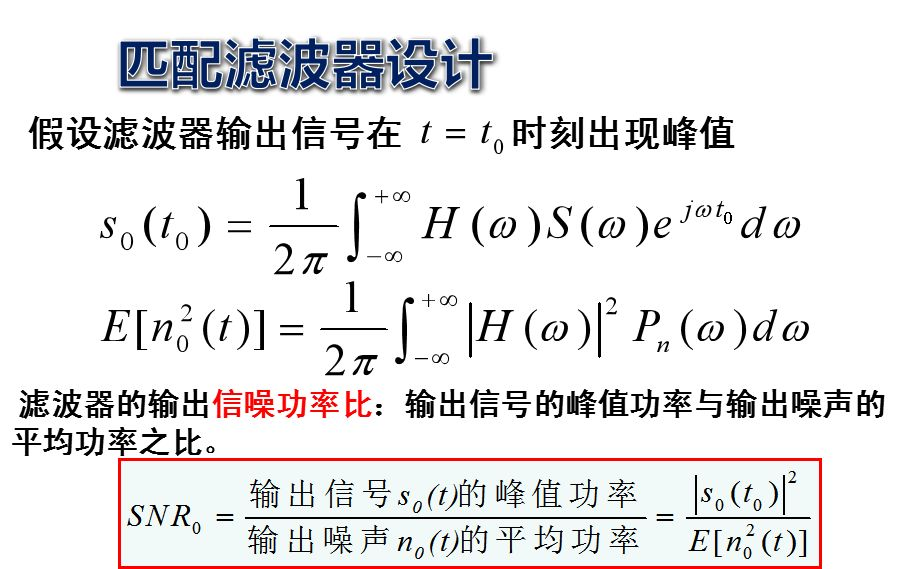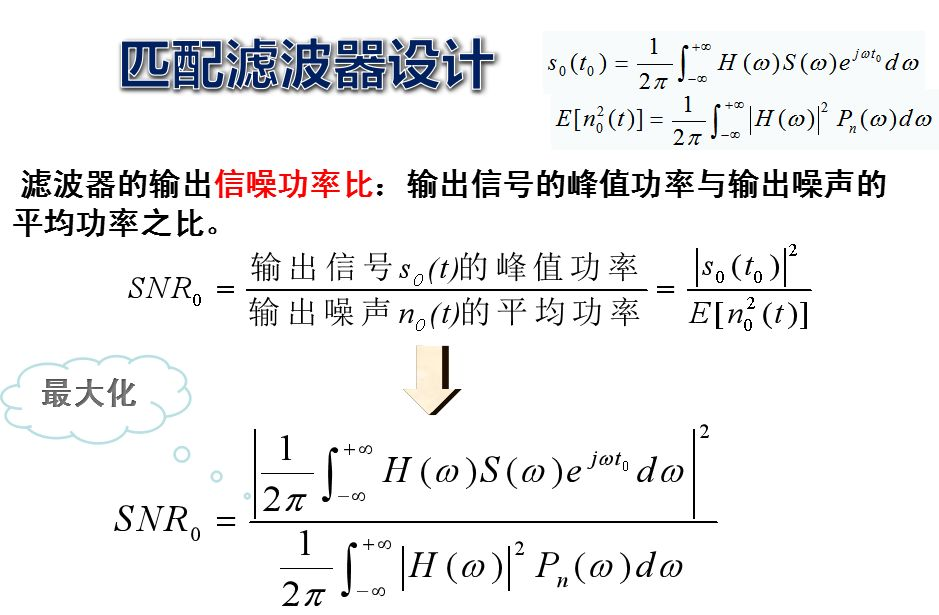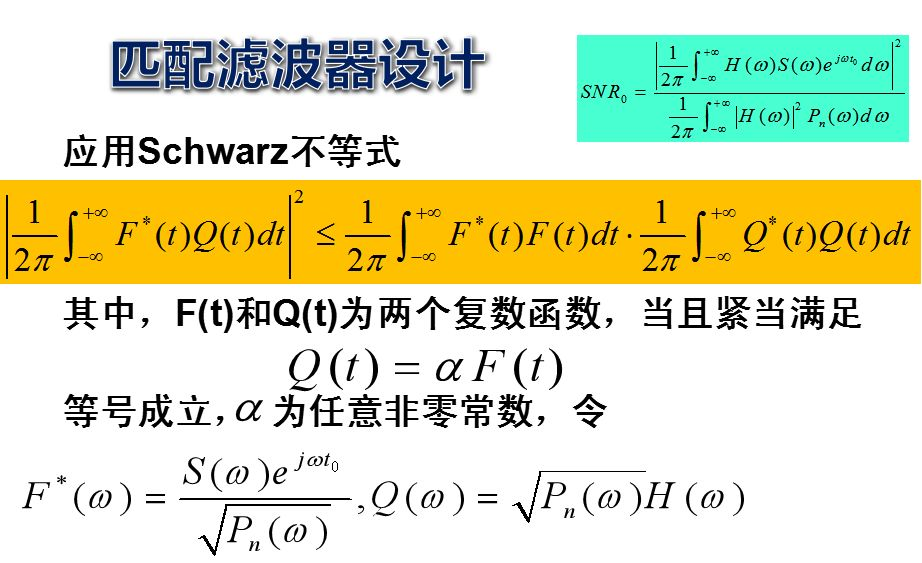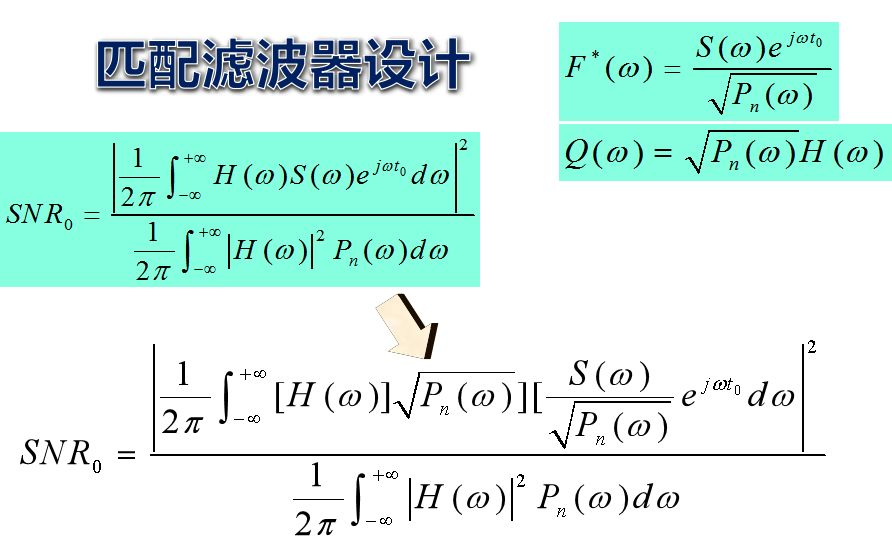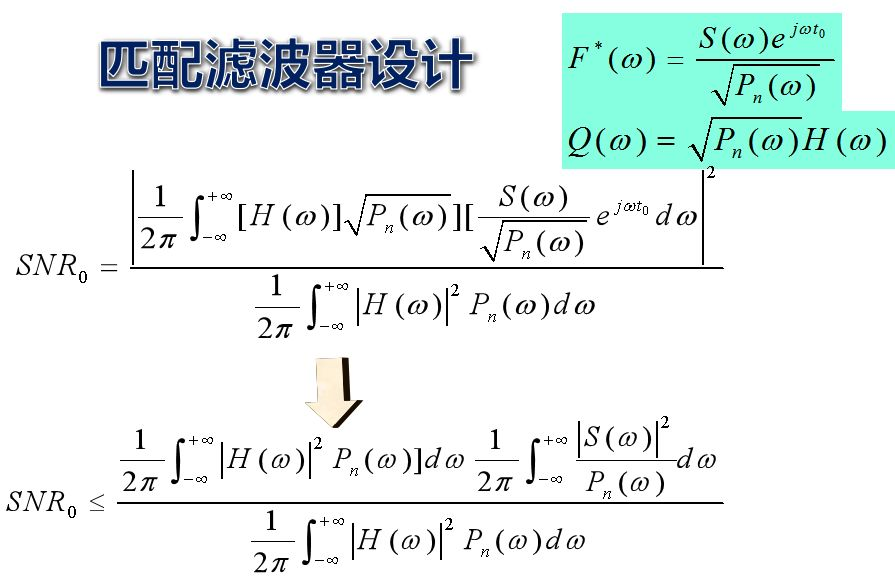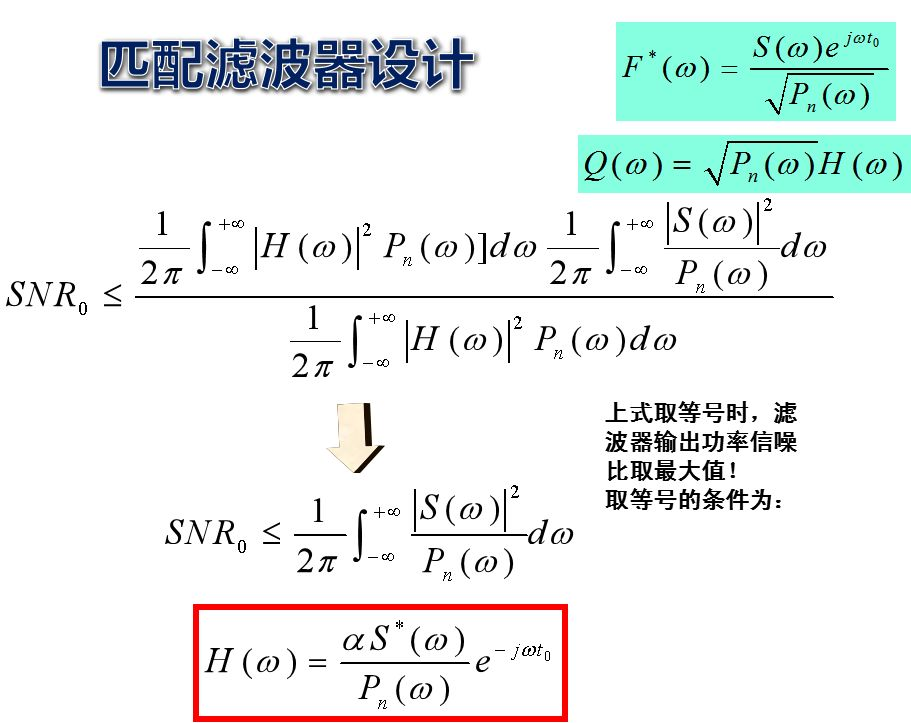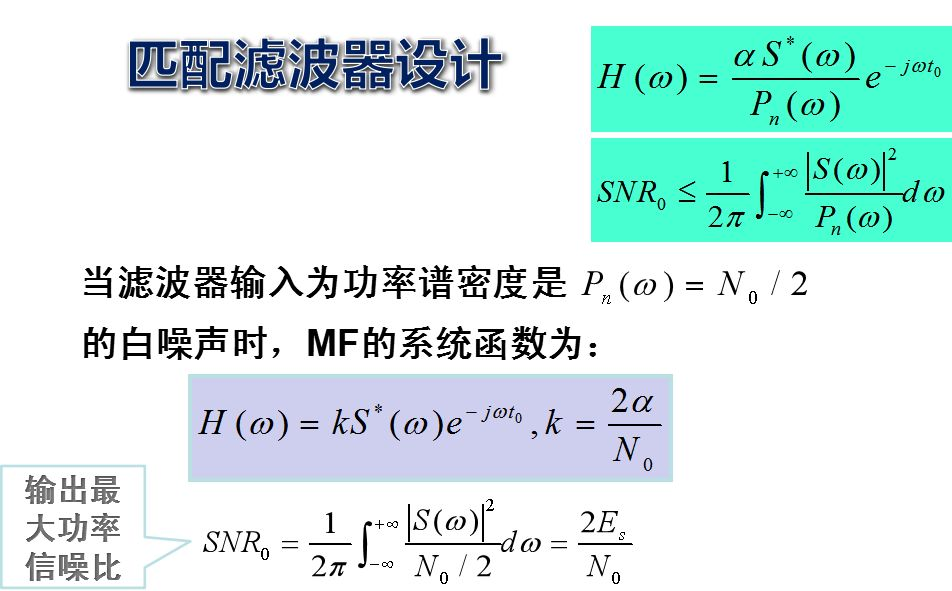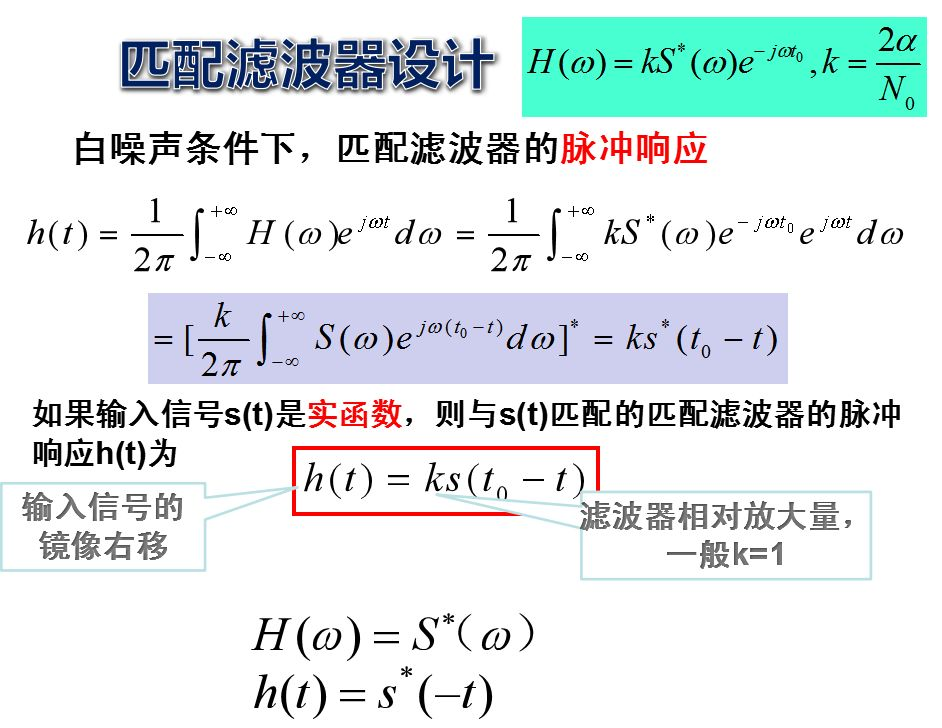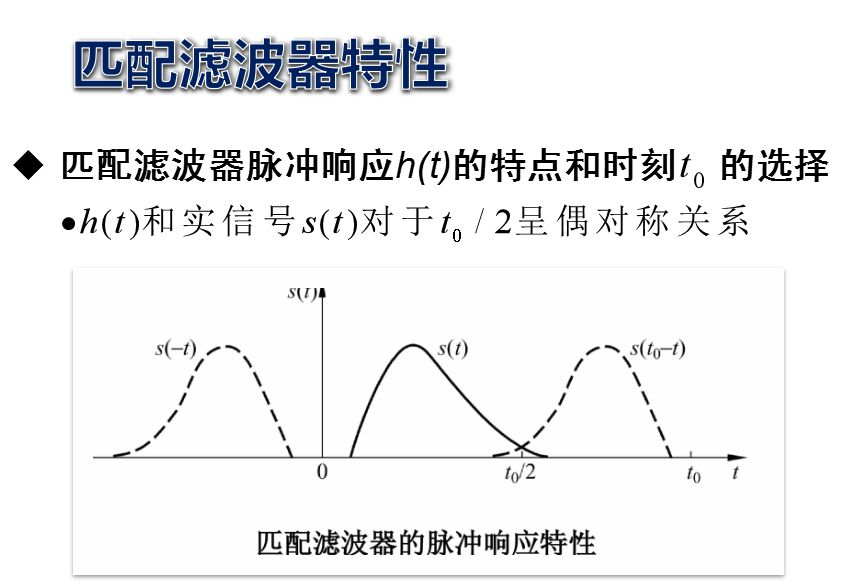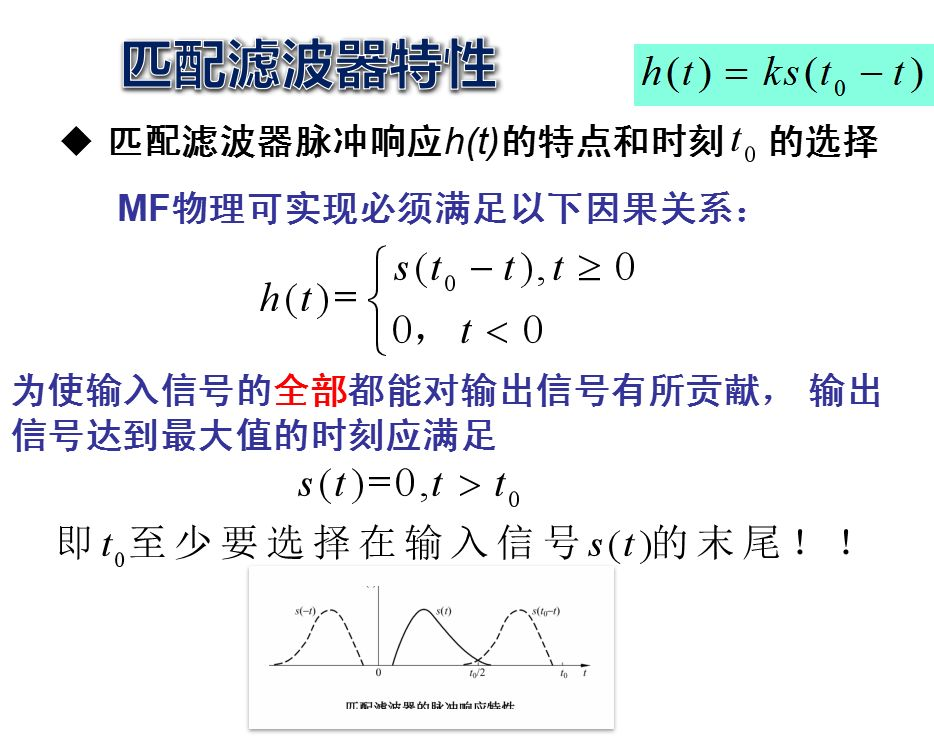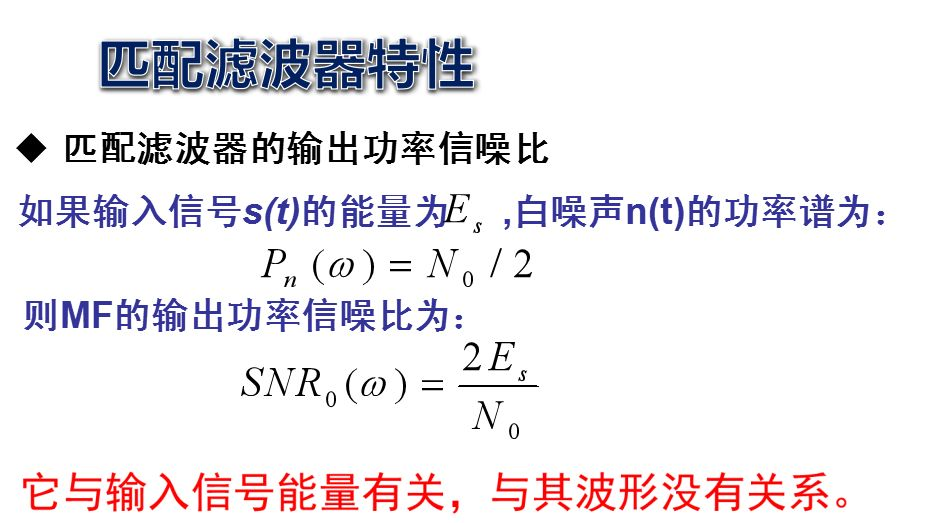在所有线性滤波器中，匹配滤波器能给出最大峰值信噪比，它只取决于输入信号能量和白噪声功率谱密度，而 与输入信号形状和噪声分布规律无关。当白噪声功率谱密度一定时，为了增大信噪比，惟一的办法是设法增大信号能量。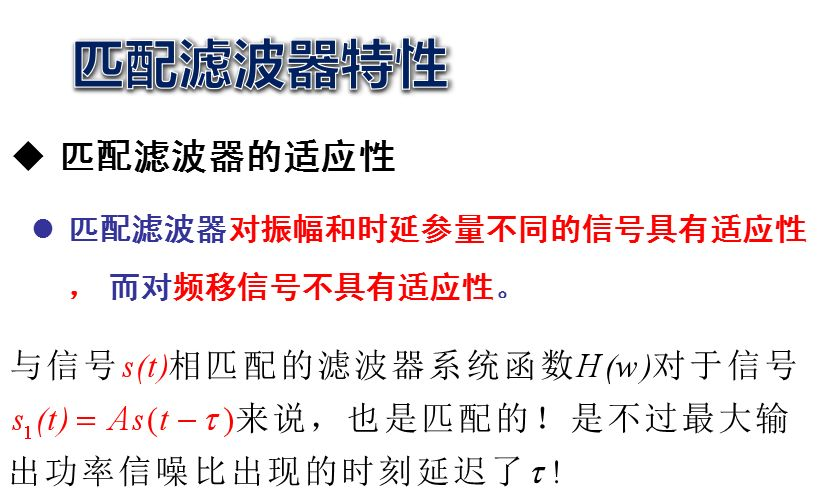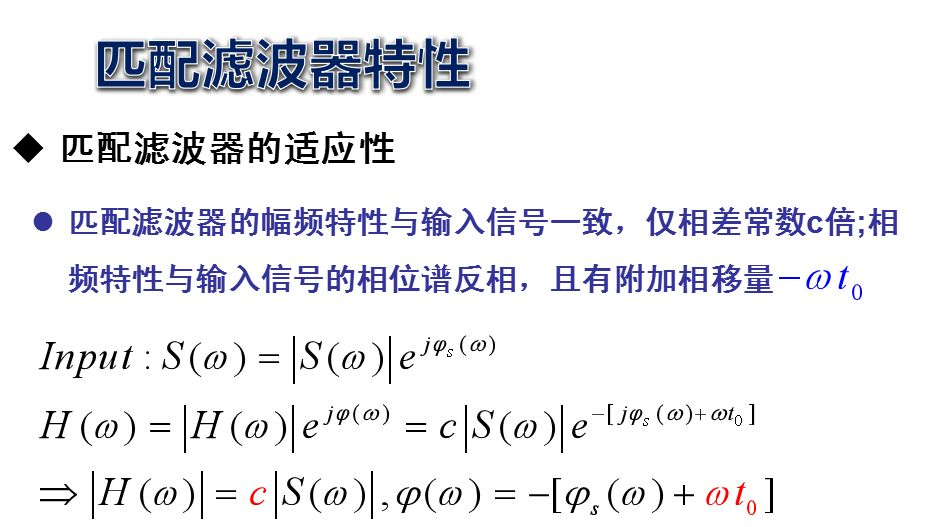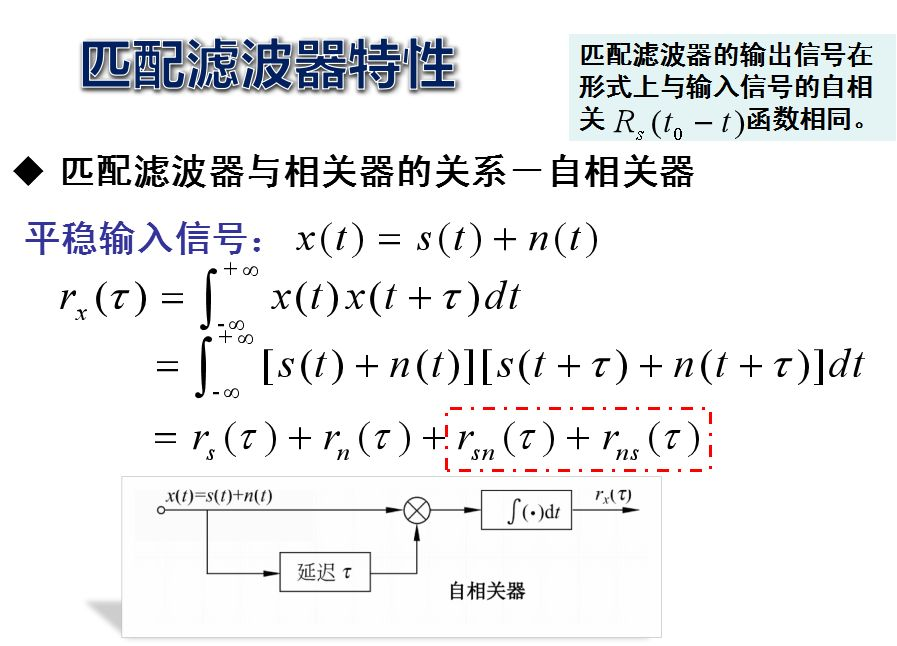实际上，匹配滤波器由其命名即可知道其鲜明的特点，那就是这个滤波器是匹配输入信号的。一旦输入信号发生了变化，原来的匹配滤波器就再也不能称为匹配滤波器了。由此，我们很容易联想到相关这个概念，相关的物理意义就是比较两个信号的相似程度。如果两个信号完全一样，不就是匹配了吗？事实上，匹配滤波器的另外一个名字就是相关接收，两者表征的意义是完全一样的。只是匹配滤波器着重在频域的表述，而相关接收则着重在时域的表述。如果输入端不存在白噪声，匹配滤波器就是一个自相关器。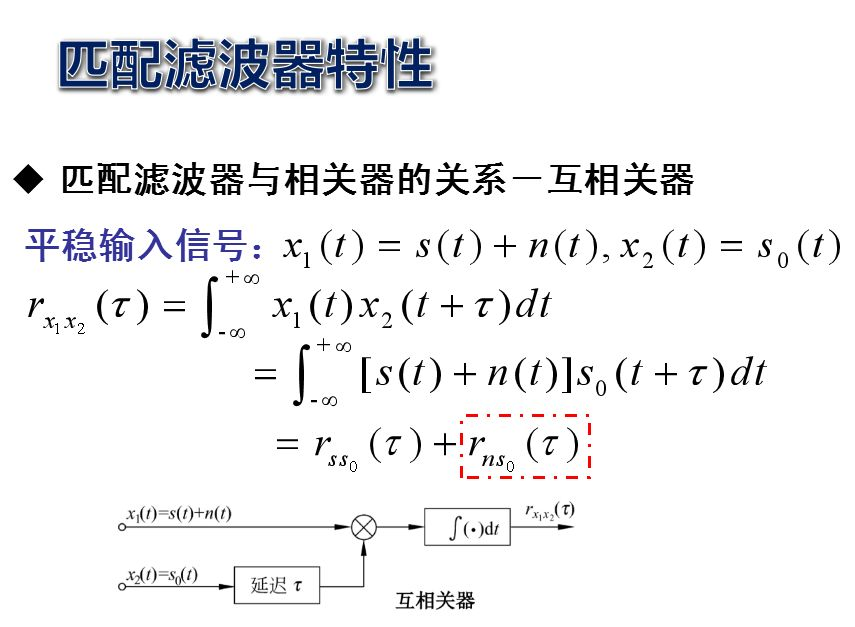在零均值白噪声条件下，匹配滤波器的输出与互相关器的输出是相等的。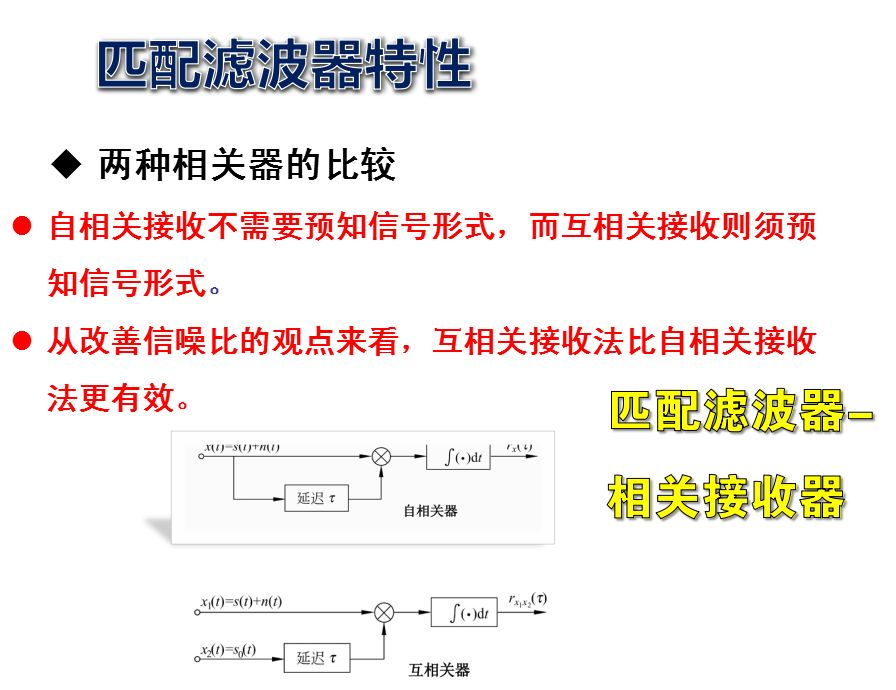自相关接收不需要预知信号形式，而互相关接收则须预知信号形式。对于收发信号在同一地点的雷达信号而言，很容易办到。但对于收发信号不在同一 地点的通信系统来说，则需要有复杂的同步系统才行。从改善信噪比的观点来看，互相关接收法比自相关接收法更有效，前者采用的参考信号是无噪声的。但当输入信噪比较大时，两种接收法的检测效果差别不大。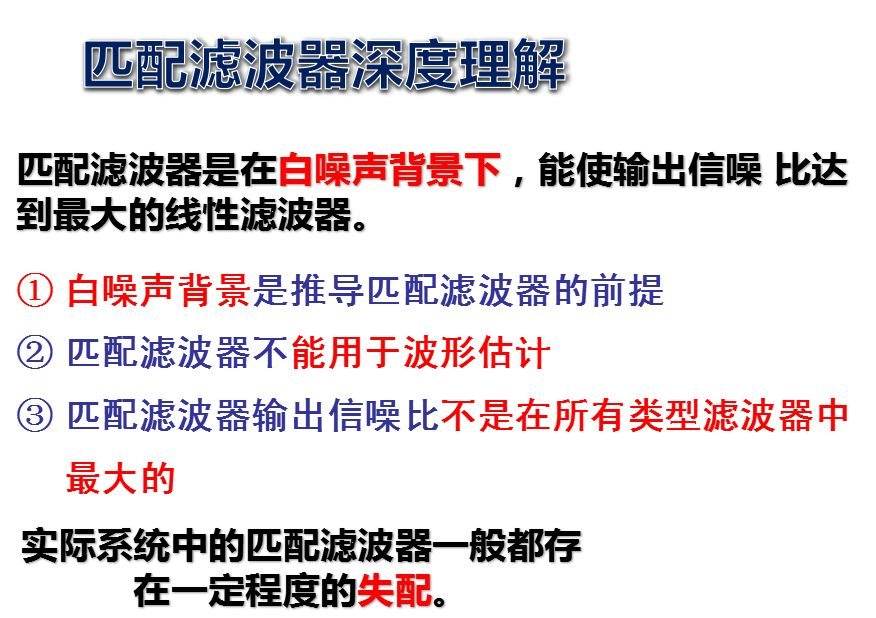白噪声背景是推导匹配滤波器的前提，但在实际应 用中，白噪声背景不是应用匹配滤波器的前提。实际系统的噪声都不完全是白噪声，但也能使用匹配滤波器，因为实际系统的噪声中白噪声所占的比例很大，一般达到90%以上，可以近似当作白噪声来处理。匹配滤波器应用的前提条件是输入信号的形式已知！匹配滤波器关心的是如何在含有噪声的信号中发现目标回波，而不关心信号波形是否失真。因此，匹配滤波器不能用于波形估计(在含有噪声的信号中除去噪声，回波信号波形)的场合，波形估计(或 称动态系统的状态估计)要用维纳滤波或Kalman 滤波等一类方法；匹配滤波器是一种线性滤波器，它的输出信噪比不是在所有类型(包括线性和非线性)滤波器中最大 的，而是在线性滤波器中能够得到最大的输出信噪比。某些情况下，非线性滤波能够得到比匹配滤波器更大的输出信噪比。对能够突破匹配滤波器的性能“极限”(对线性滤波器而言)的非线性滤波器， 是目前信号检测领域的研究热点之一，它对雷达提 高对RCS很小的隐身目标的探测能力，全面提高雷达系统的探测威力意义重大。在雷达系统中，由于目标反射的调制作用，目标的回波不仅在包络上会出现调制(低频调制)，而且由于目标运动会带来多普勒调制，使回波信号附加一个多普勒频移。匹配滤波器一定程度的失配会使滤波性能损失一点，但这并不妨碍匹配滤波器的使用。在通信系统中引起匹配滤波器失配的主要原因就是信道衰减，这种衰减可能对不同频率成分的信号衰减程 度不一样，就像雷达目标回波中的包络一样会出现低频调制，但这种调制带来的影响比目标回波的多普勒频移要小的多。因此，理论上通信系统中的匹配滤波效果比雷达系统更好。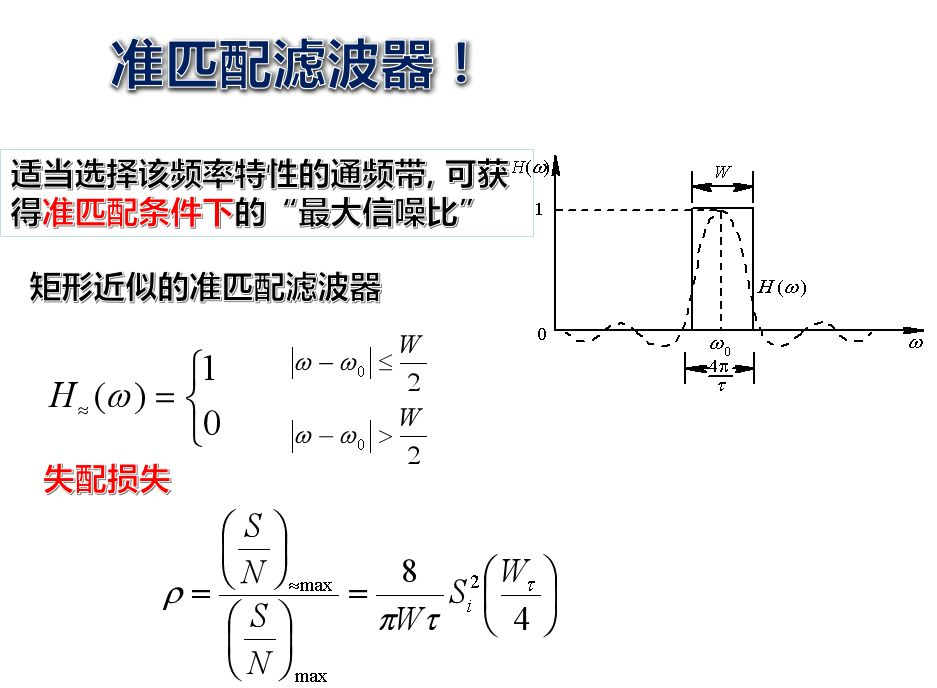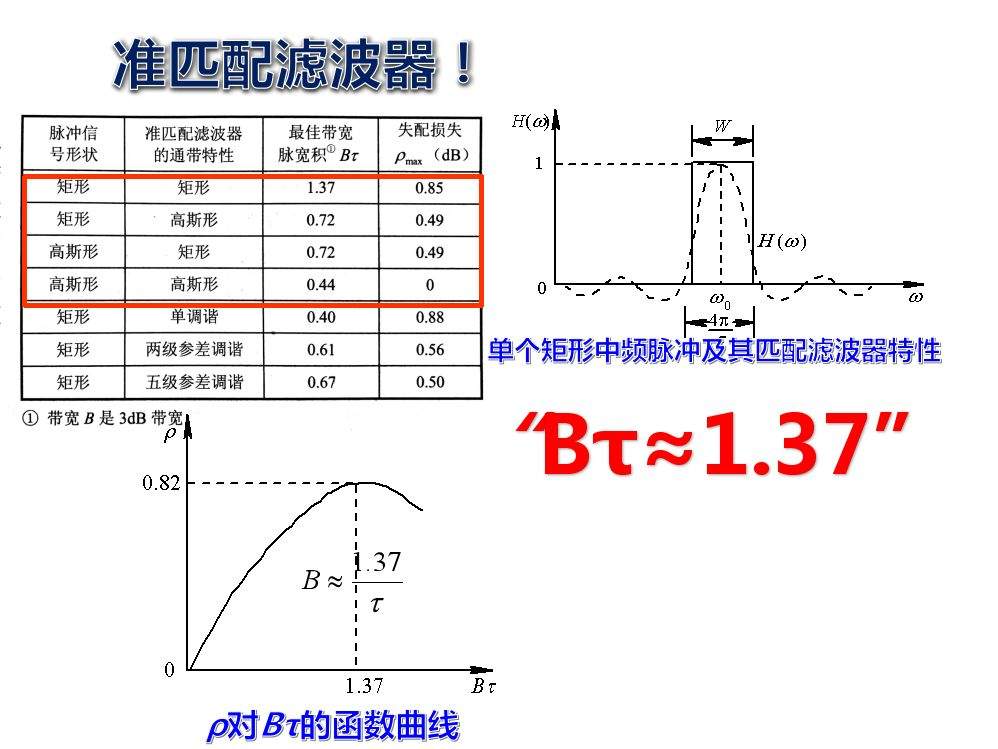展开全文• 文章目录1、采用匹配滤波器的二进制基带传输系统误码性能分析1.2 系统模型2、带宽无限信道二进制匹配滤波基带系统误码率3、带宽有限信道二进制匹配滤波基带系统误码率4、多进制匹配滤波基带系统误码率   这部分...
• ## 成形滤波器和匹配滤波器

万次阅读 多人点赞 2017-07-31 10:36:15
为了消除码间串扰，根据奈奎斯特第一准则，分析用平方根升余弦滚降滤波器来想实现无码间干扰。 以下摘自通信原理--樊昌信--P151 该理想滤波器，物理不可实现，还需要寻找另外的设计----- 为了解决理想低通...
• 匹配滤波器捕获的基本原理进行了研究并着重讨论了其多种FPGA实现结构。　关键词：直接序列扩频；数字匹配滤波器；折叠滤波；部分相关　20世纪80年代末90年代初，直接序列扩频码分多址（DS/CDMA）开始进入商用，...直接序列扩频
• 首先介绍了数字匹配滤波器原理，然后阐述了多模式DMF的设计原理，在同一个直接序列扩频通信系统的接收端集成多种模式，实现对多种扩频比扩频信号的解扩，提高通信系统的性能。在此基础上，通过MATLAB仿真验证其...
• 多用户检测中匹配滤波器的FPGA实现，赵彦龙，李艳萍，首先简要介绍匹配滤波器在多用户检测中的作用，以及多用户检测匹配滤波器的基本结构和工作原理，在此基础上提出了一种基于FPGA(Fiel
• 多用户检测中匹配滤波器的研究与实现，赵彦龙，李艳萍，首先简要介绍匹配滤波器在多用户检测中的作用，以及多用户检测匹配滤波器的基本结构和工作原理，在此基础上提出了一种基于FPGA(Fiel
• 基于FPGA的匹配滤波器实现。有具体的原理图设计。以及quartus工程
• 数字匹配滤波器的优化设计方案及在FPGA上的实现，刘贤福，，本文对数字匹配滤波器原理和结构进行了简要的介绍，在扩频通信中，应用数字匹配滤波器实现m序列同步,并详细讨论了其基于FPGA(现��...